# Selina Solutions Concise Maths Class 7 Chapter 5: Exponents (Including Laws of Exponents)

Selina Solutions Concise Maths Class 7 Chapter 5 Exponents (Including Laws of Exponents) are designed by a set of highly experienced faculty at BYJU’S. The solutions contain explanations in simple language to improve analytical thinking among students. PDF of solutions are available for free download to boost their exam preparation. Selina Solutions Concise Maths Class 7 Chapter 5 Exponents (Including Laws of Exponents), PDF links are provided here.

Chapter 5 helps students understand the exponential form of a number, laws of exponents and more about indices. Students can refer to the solutions PDF and solve the chapter wise problems on a daily basis, for a better academic score.

## Selina Solutions Concise Maths Class 7 Chapter 5: Exponents (Including Laws of Exponents) Download PDF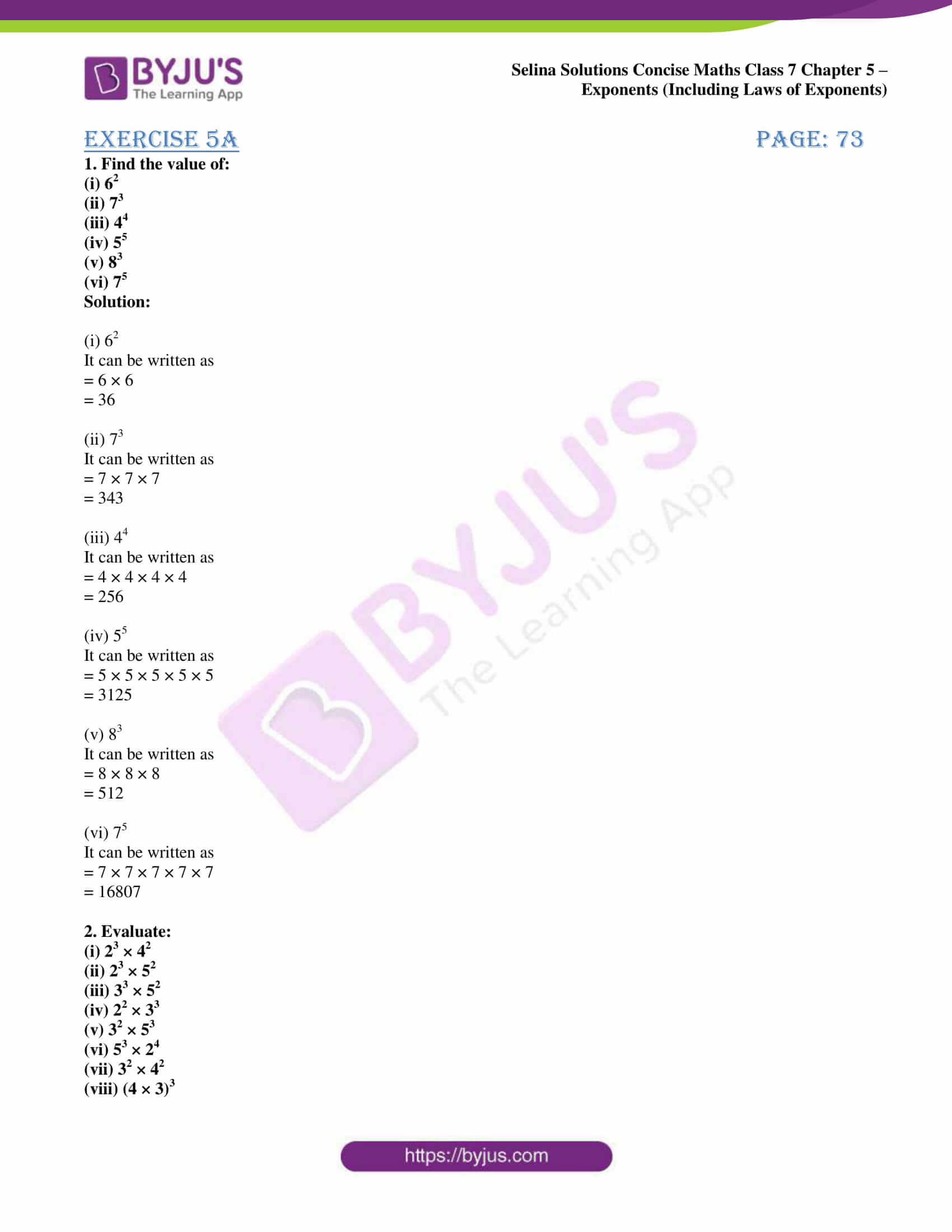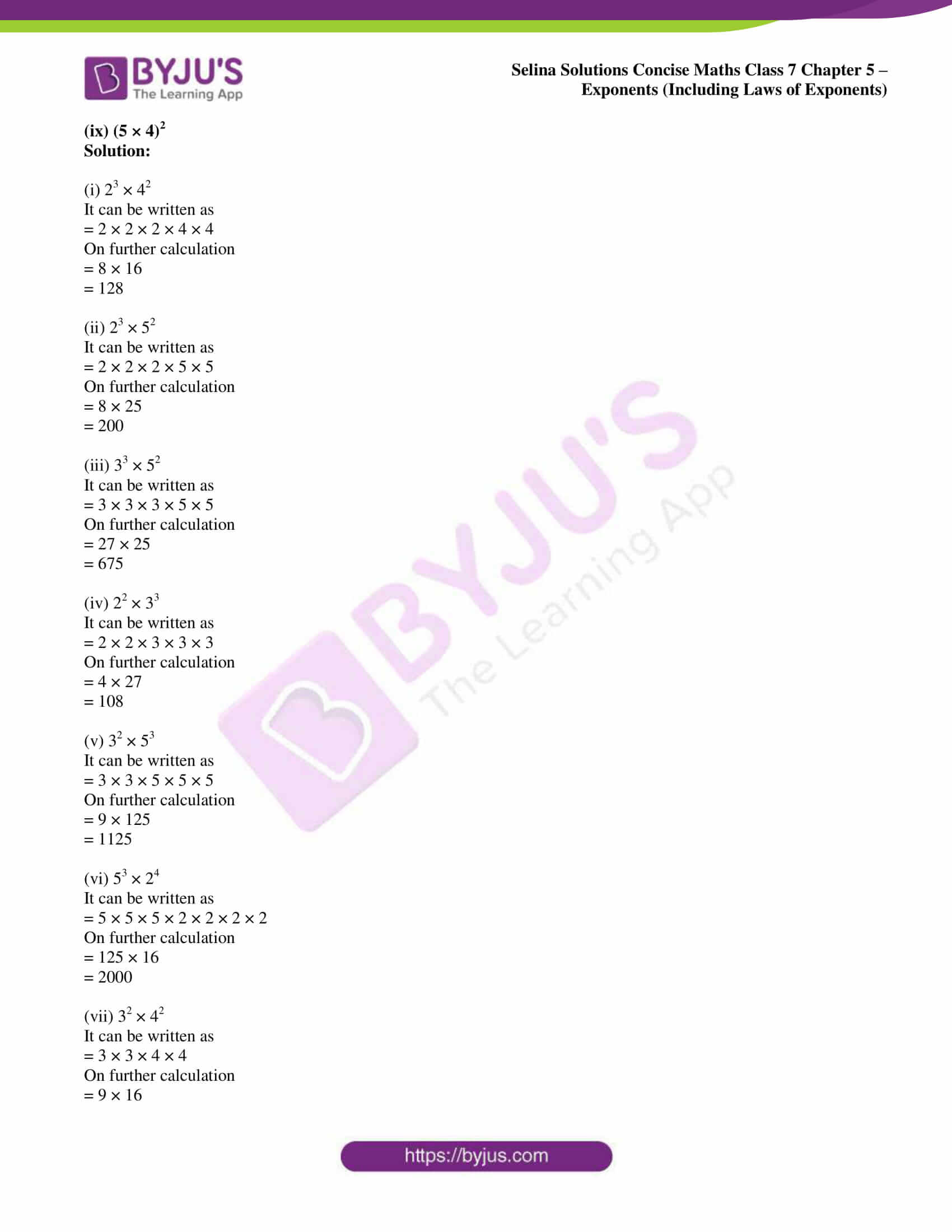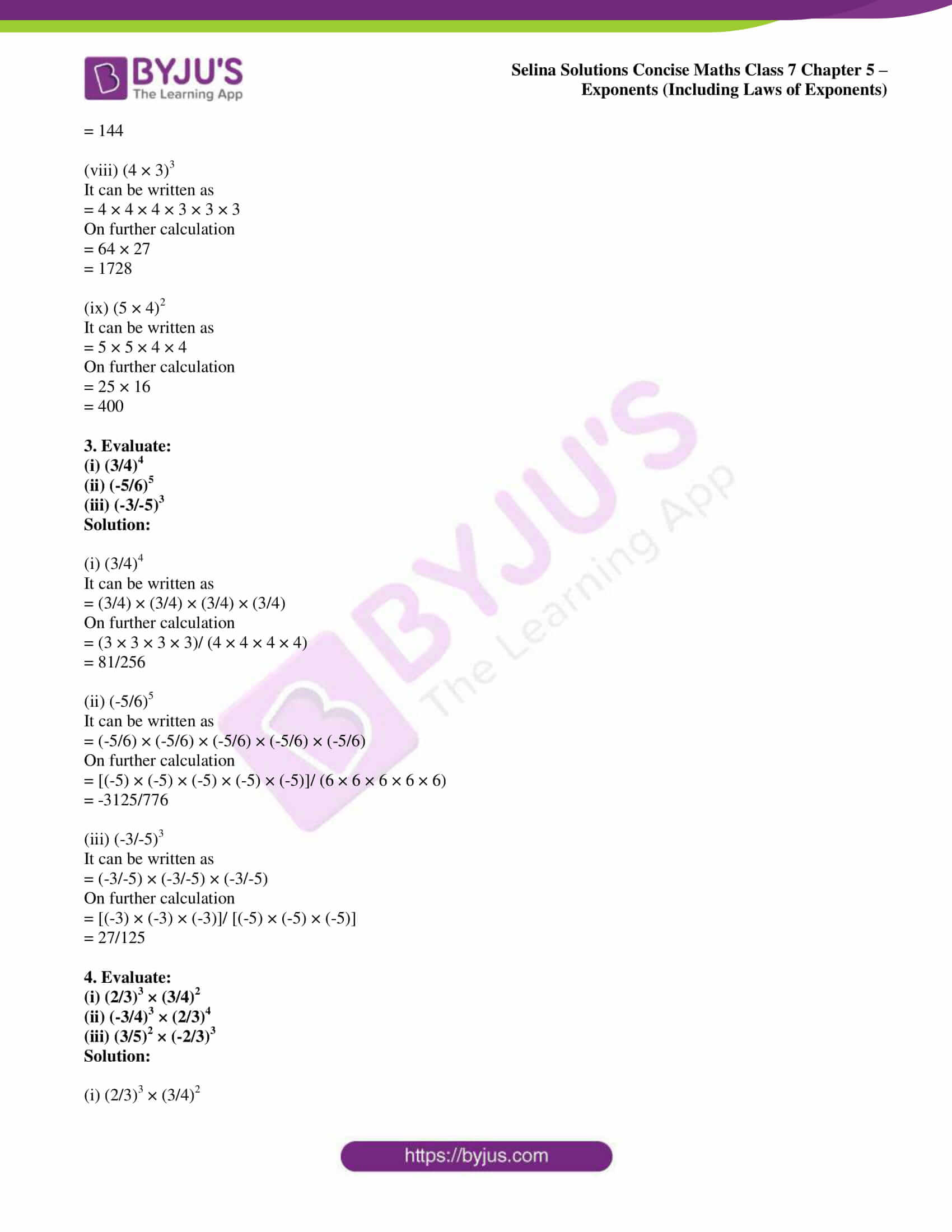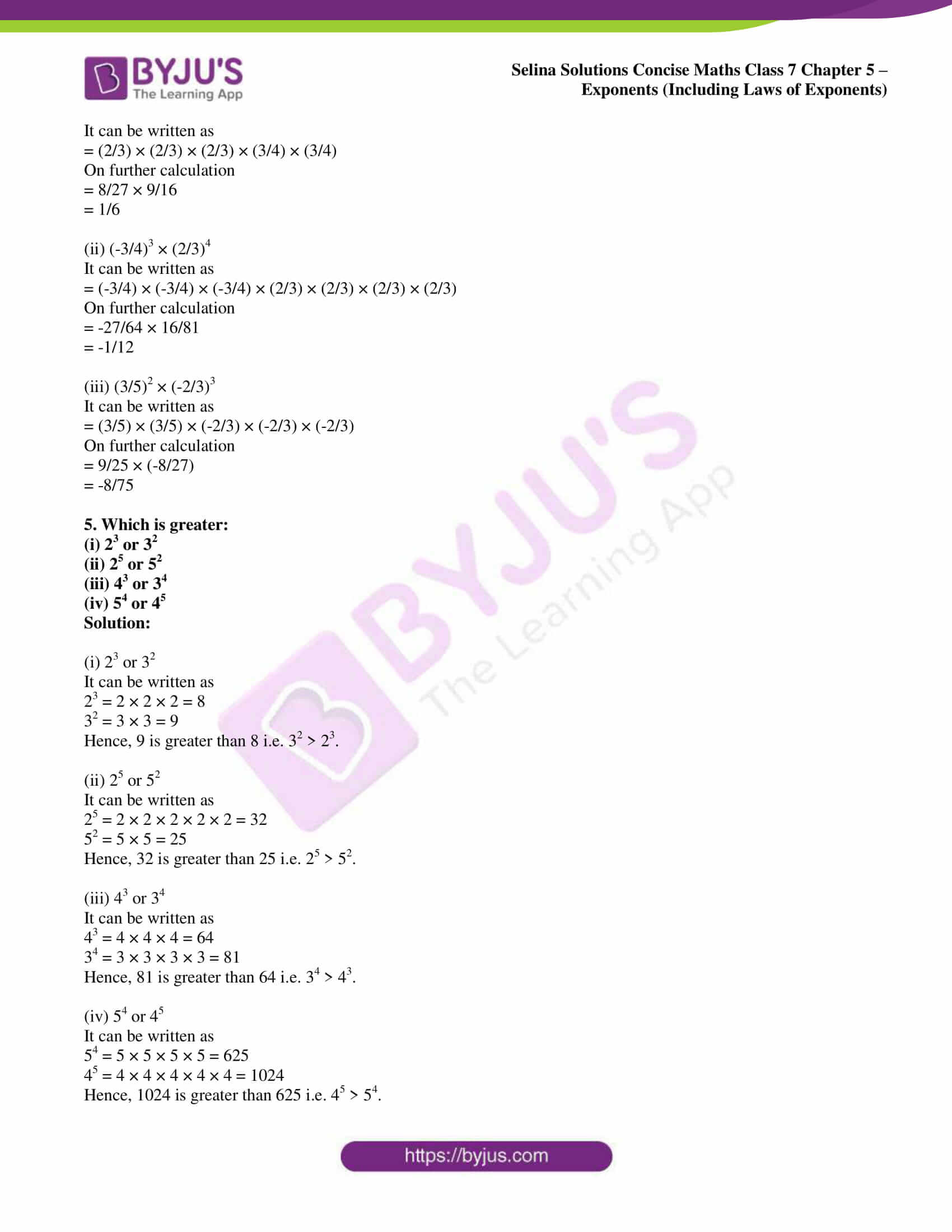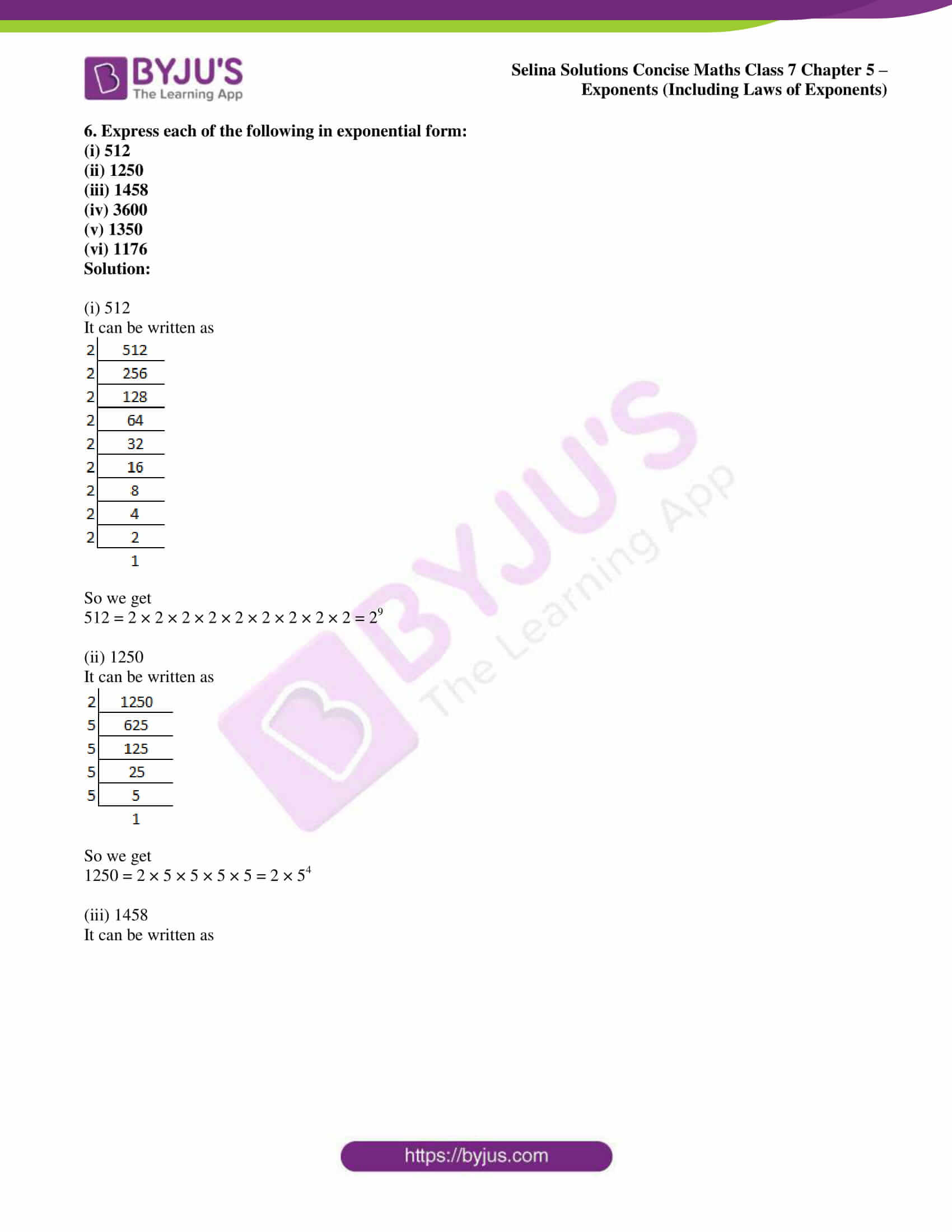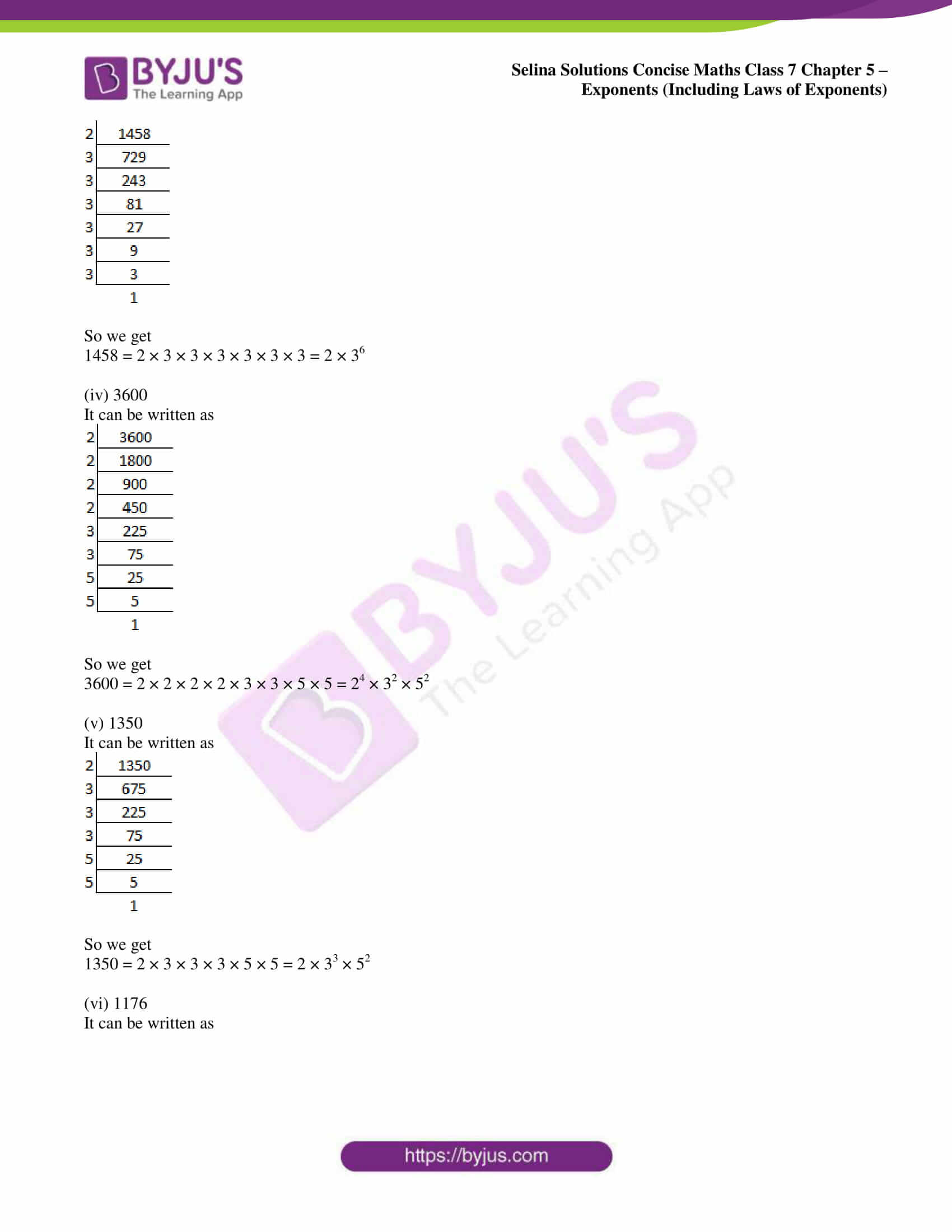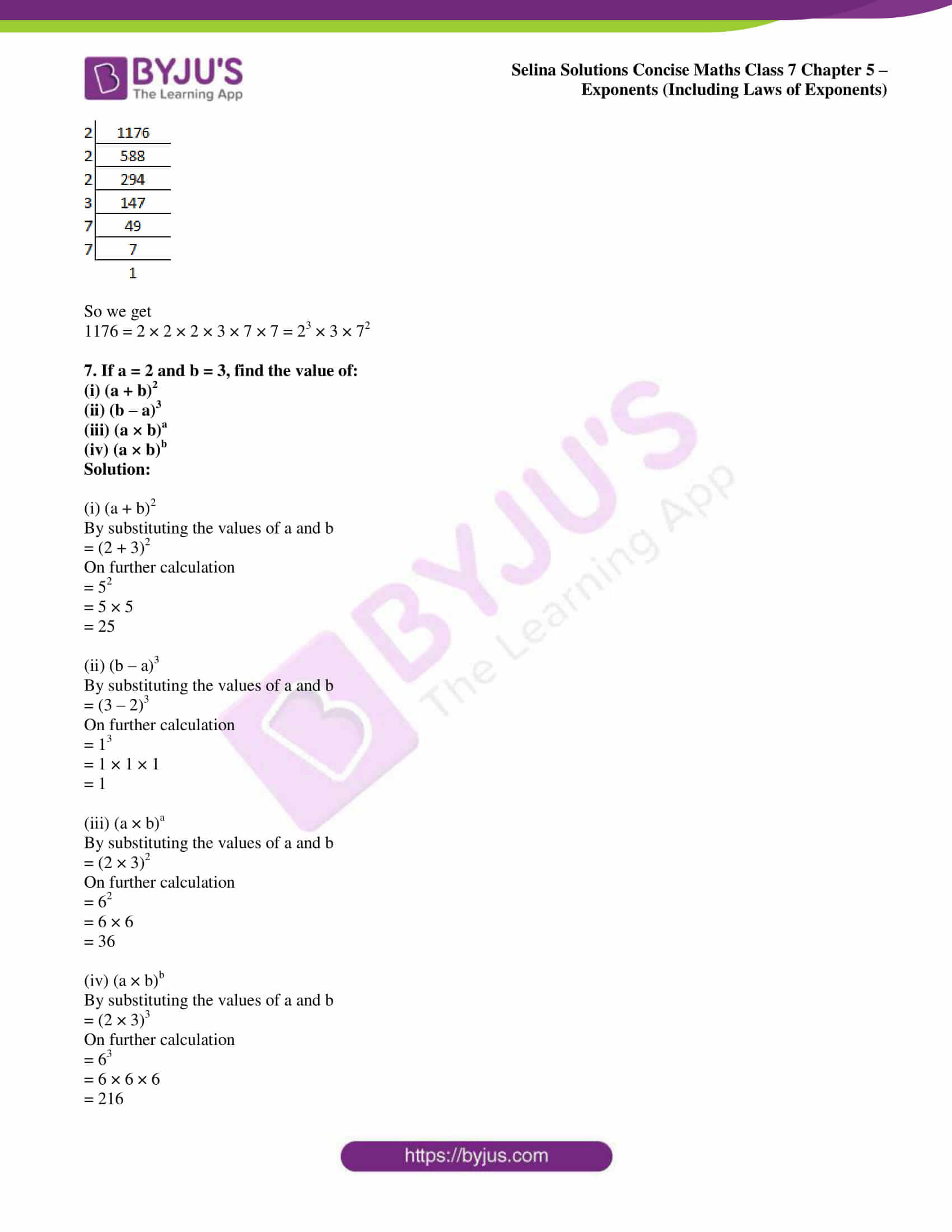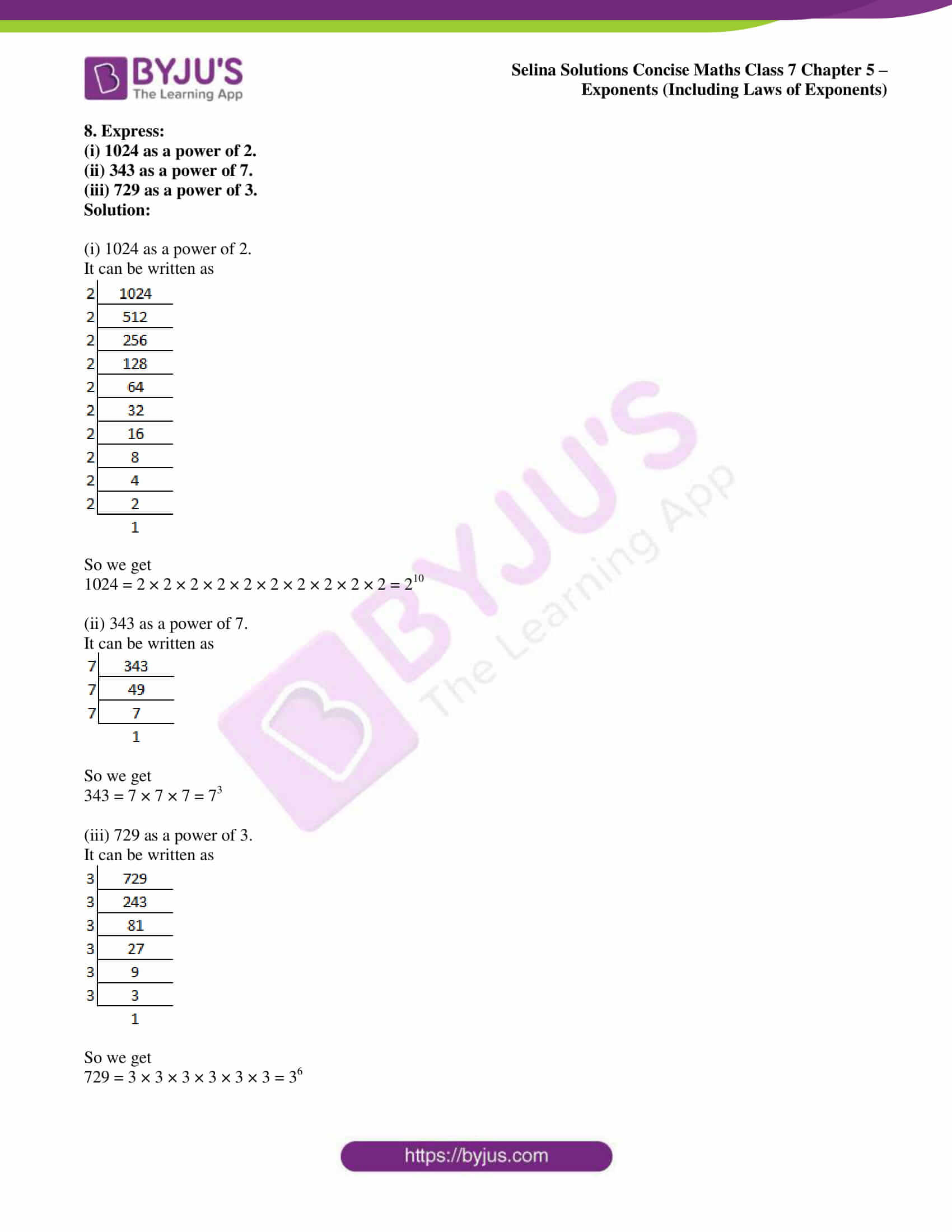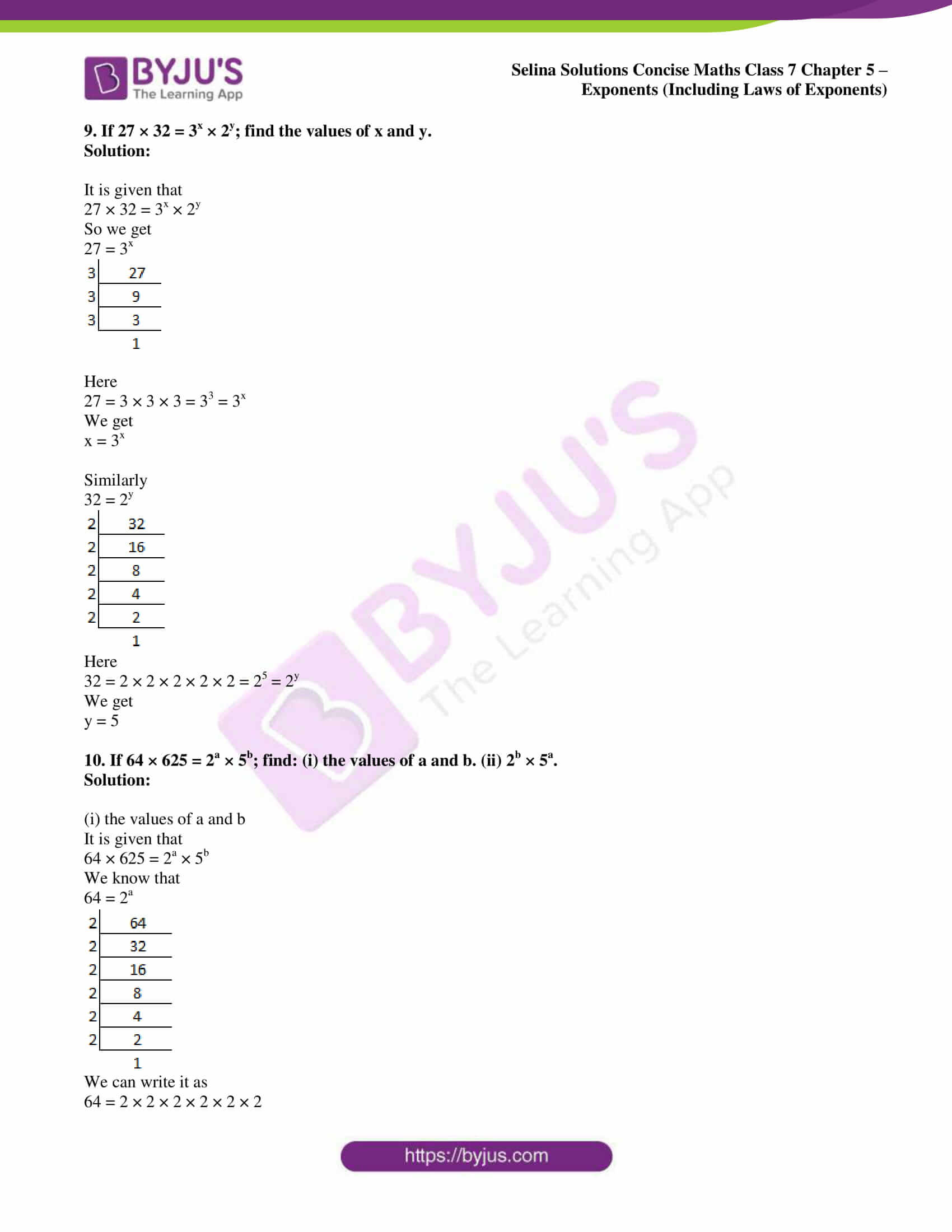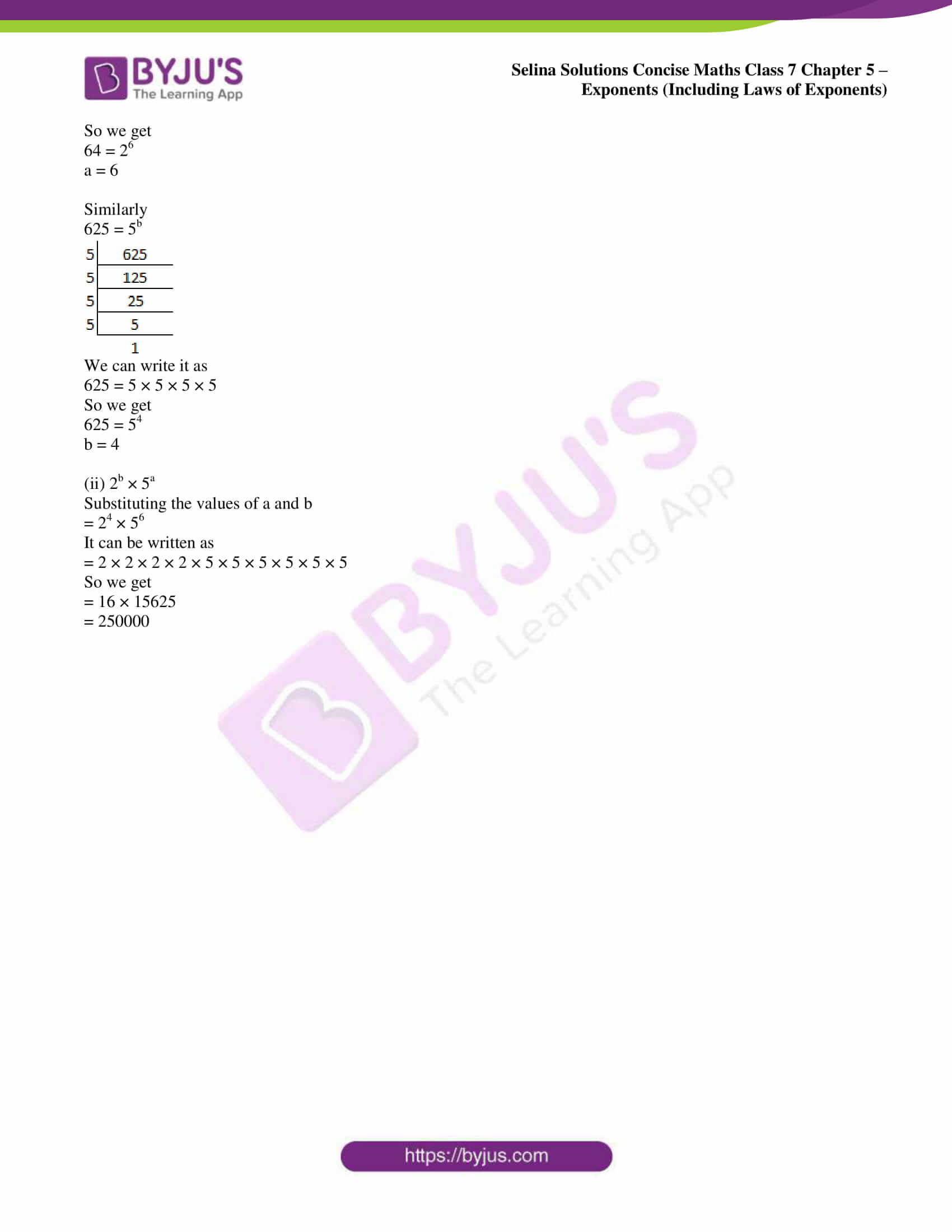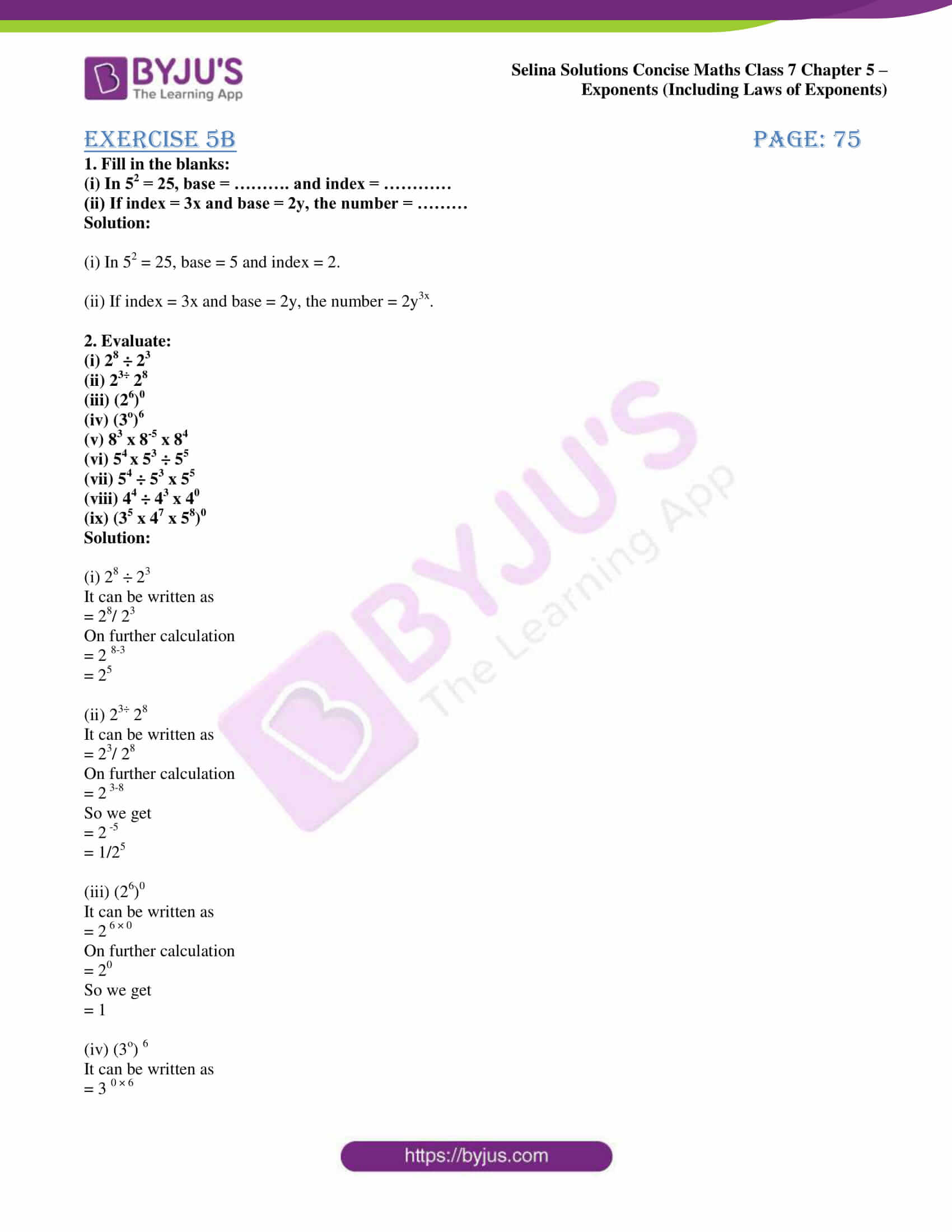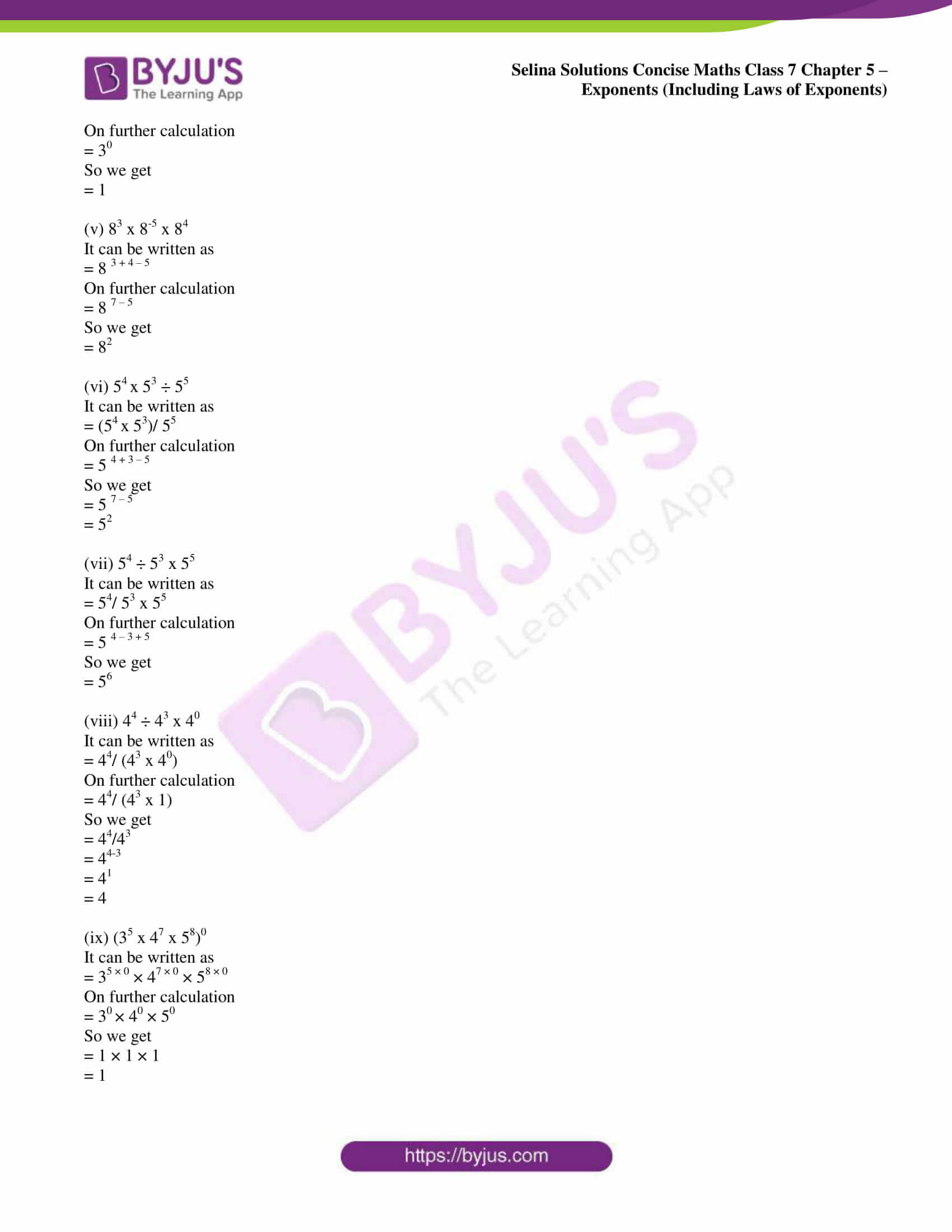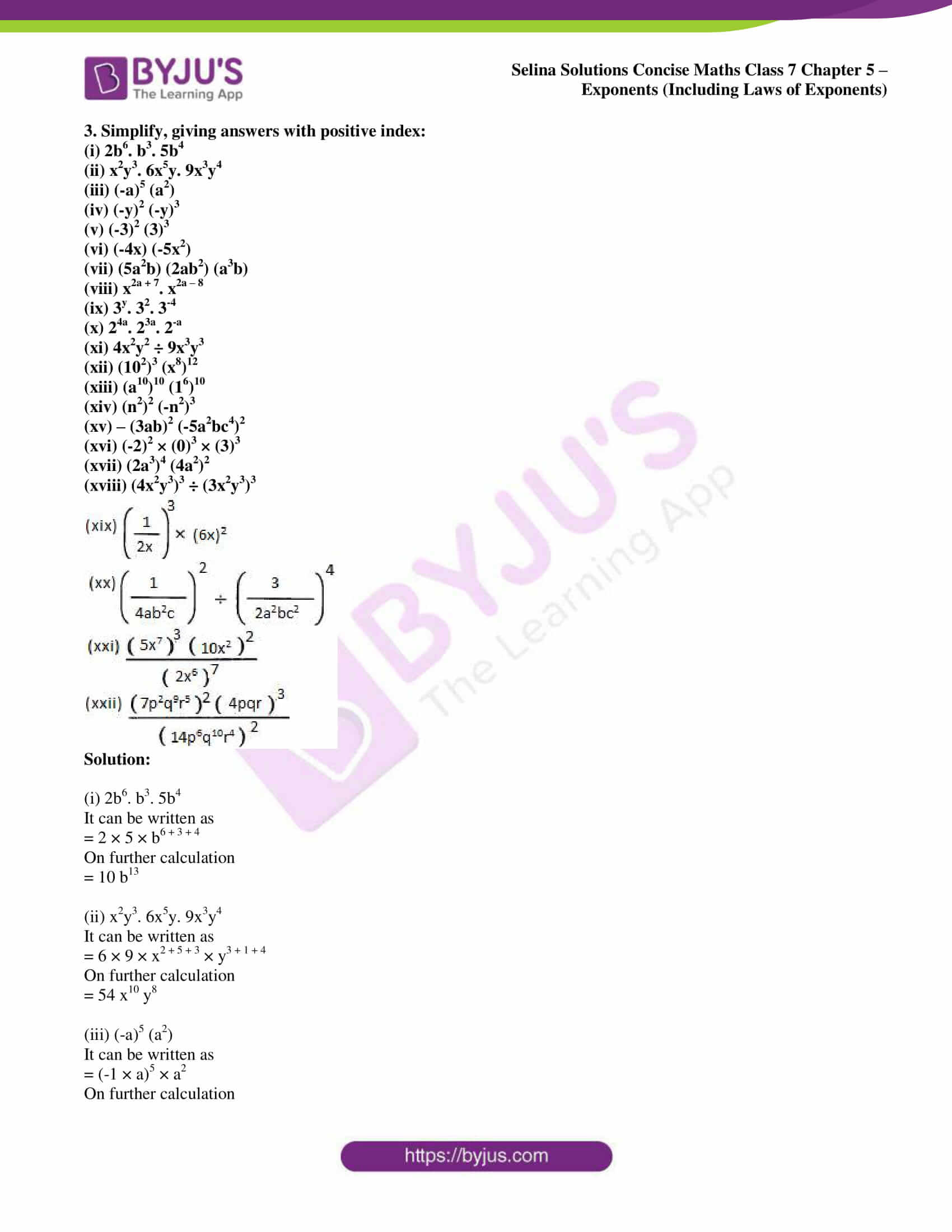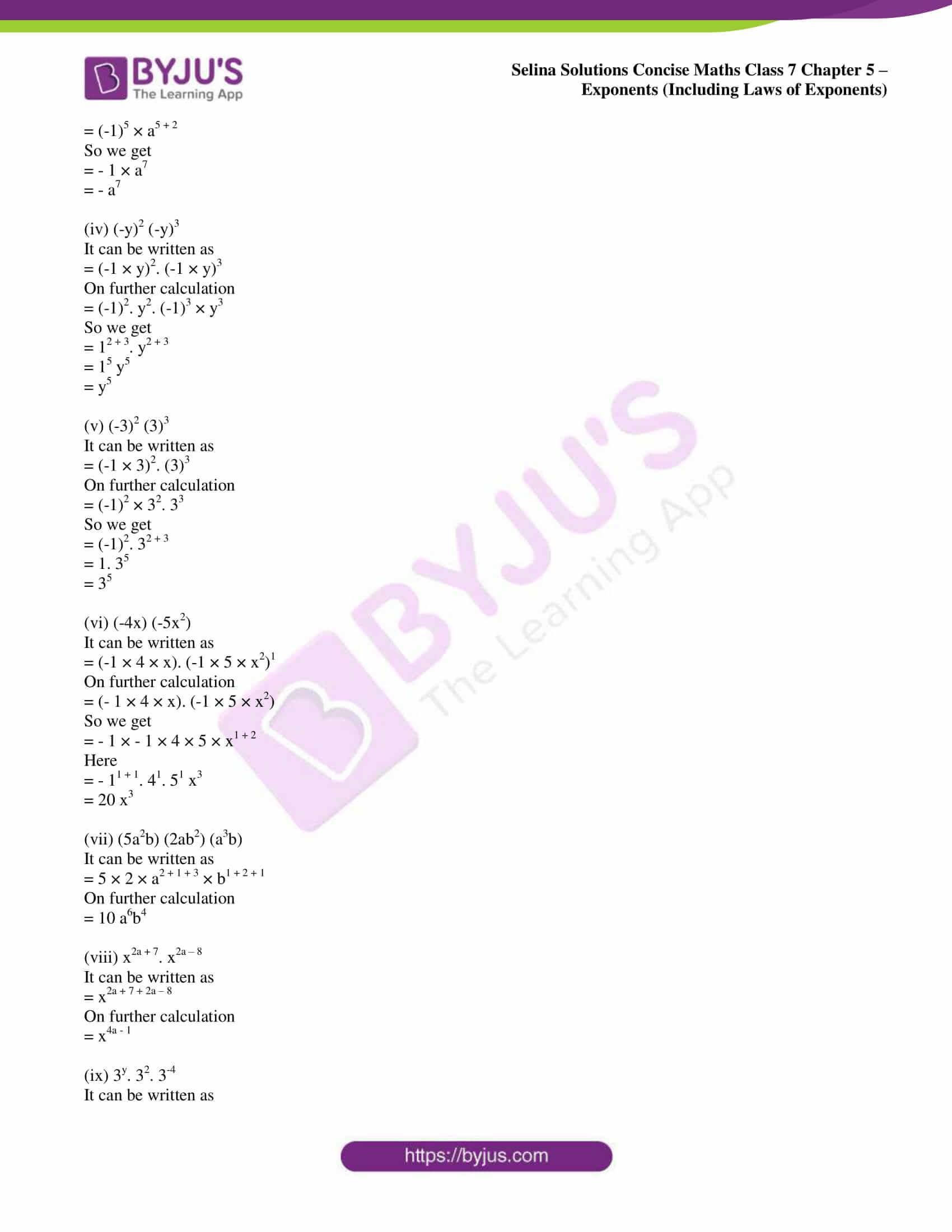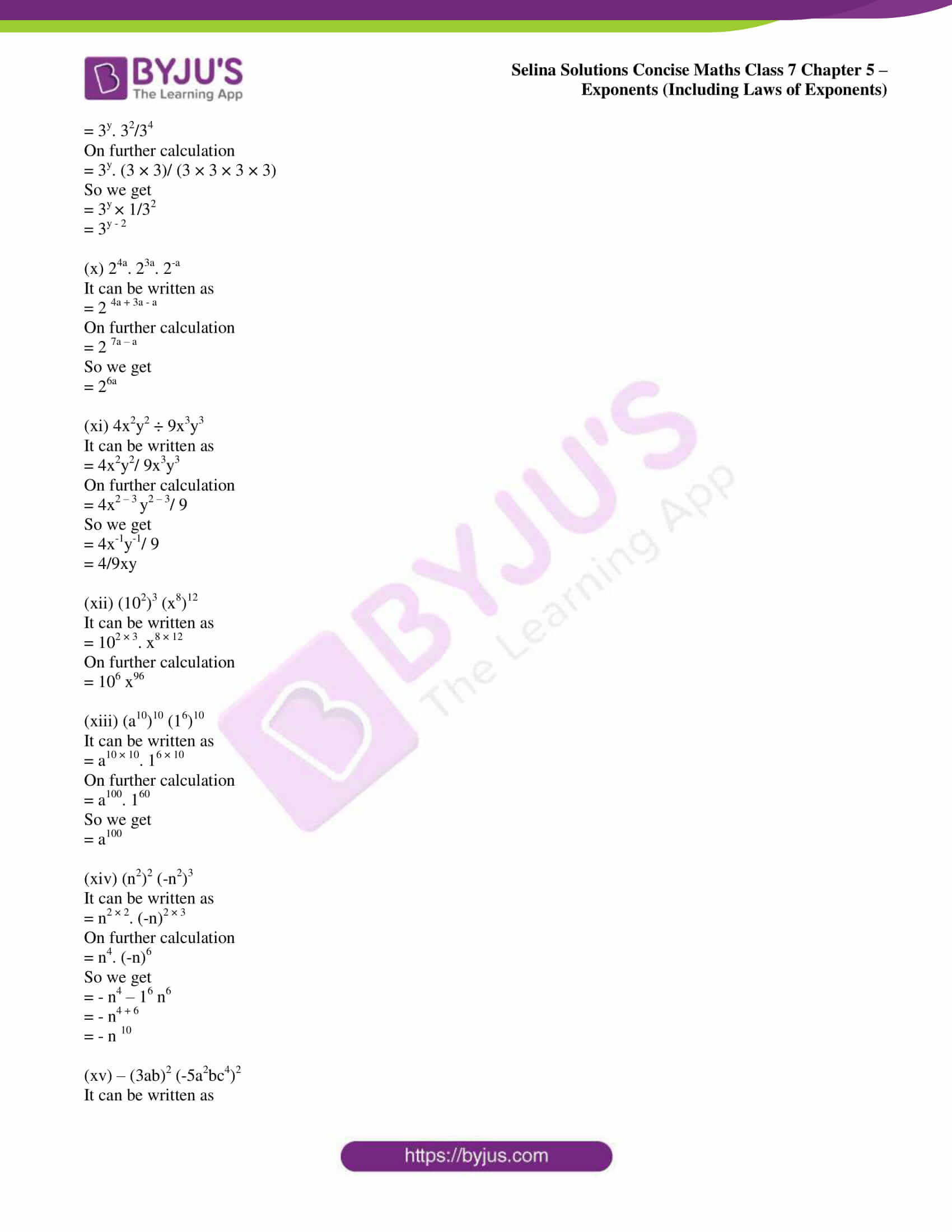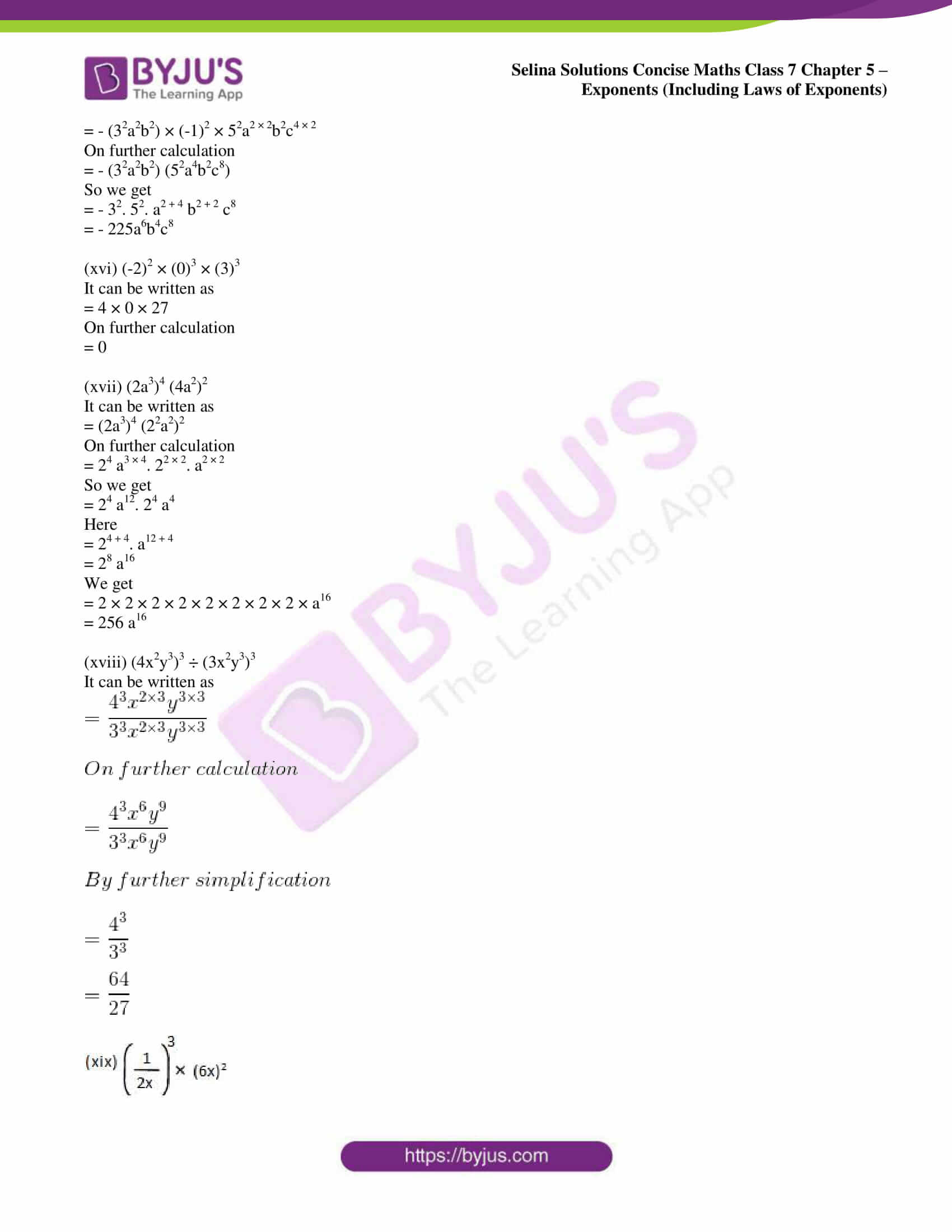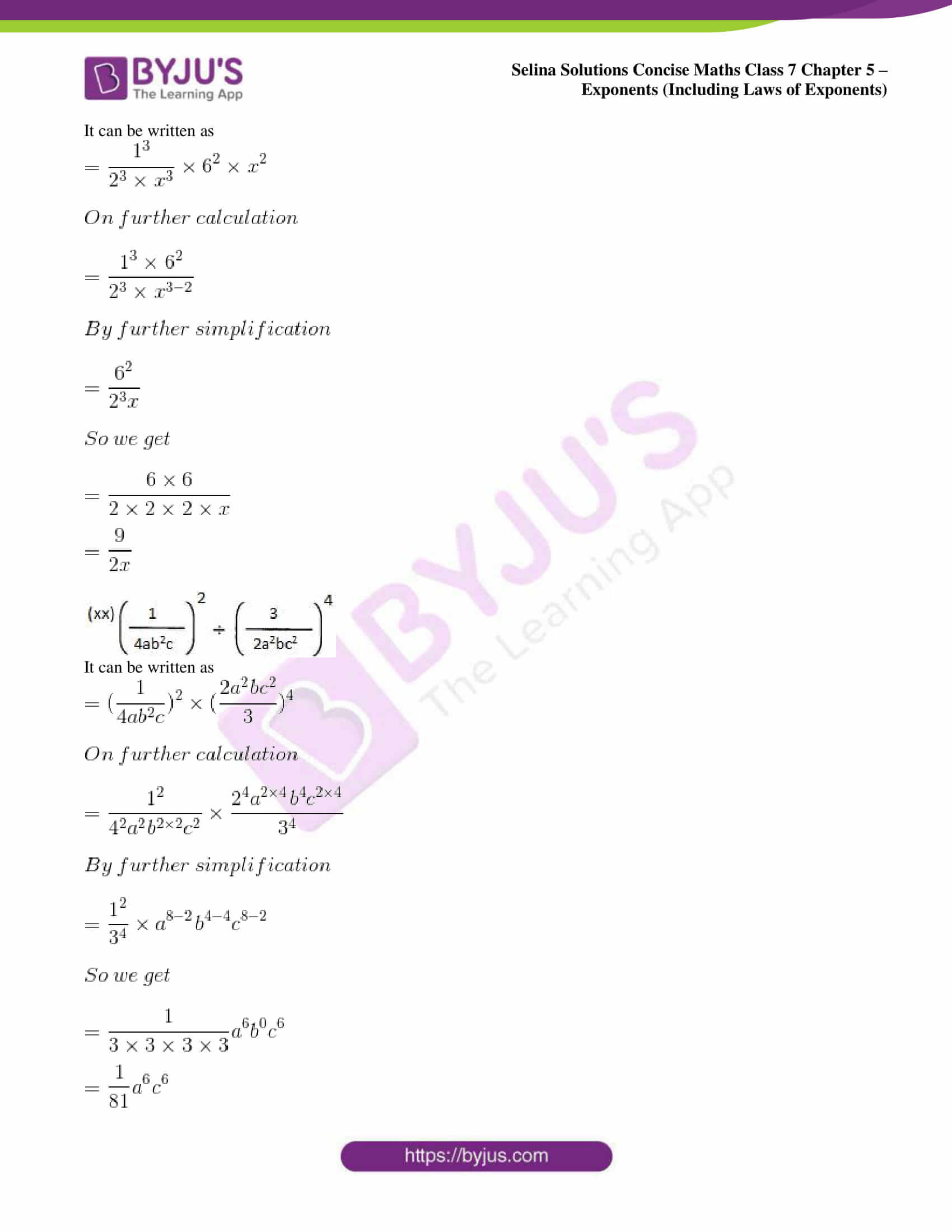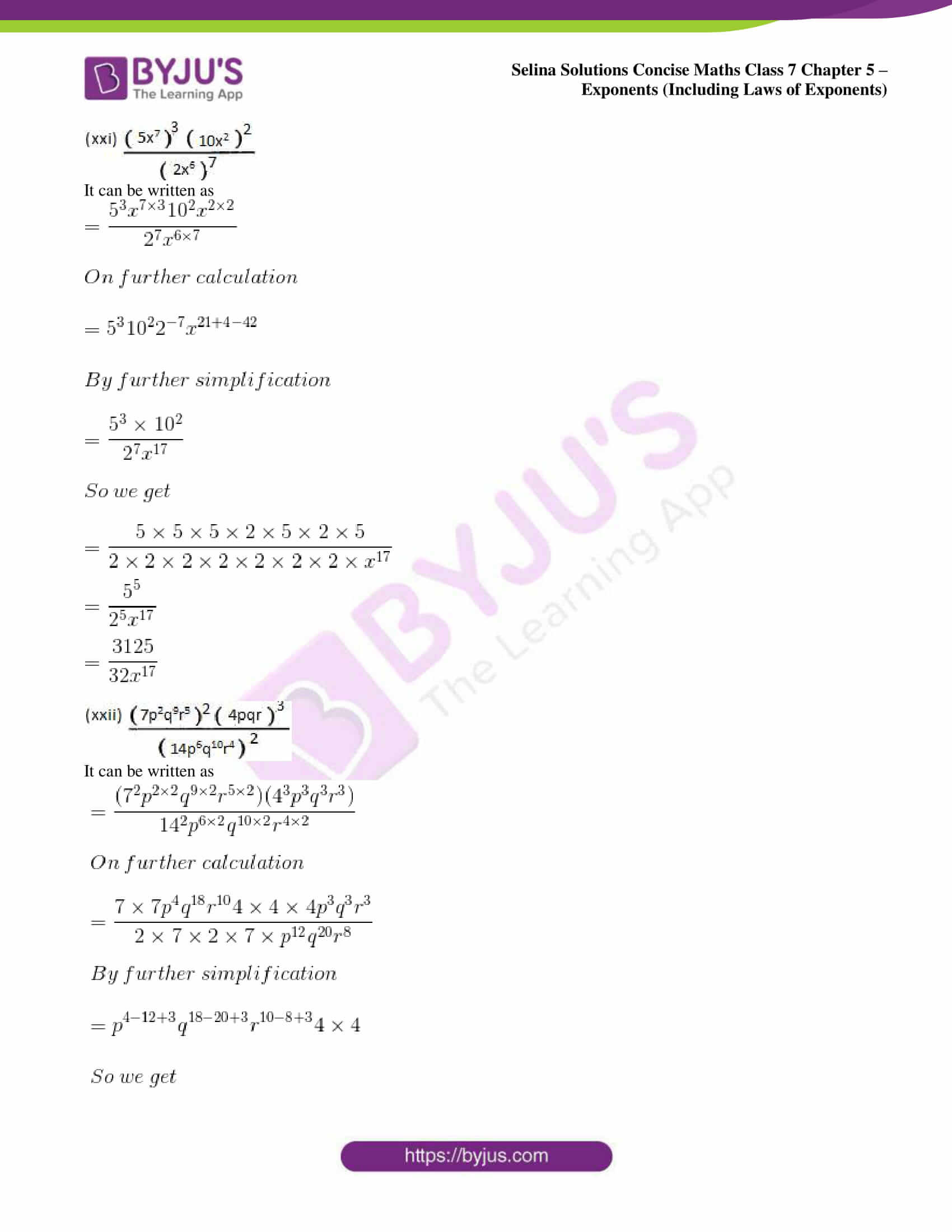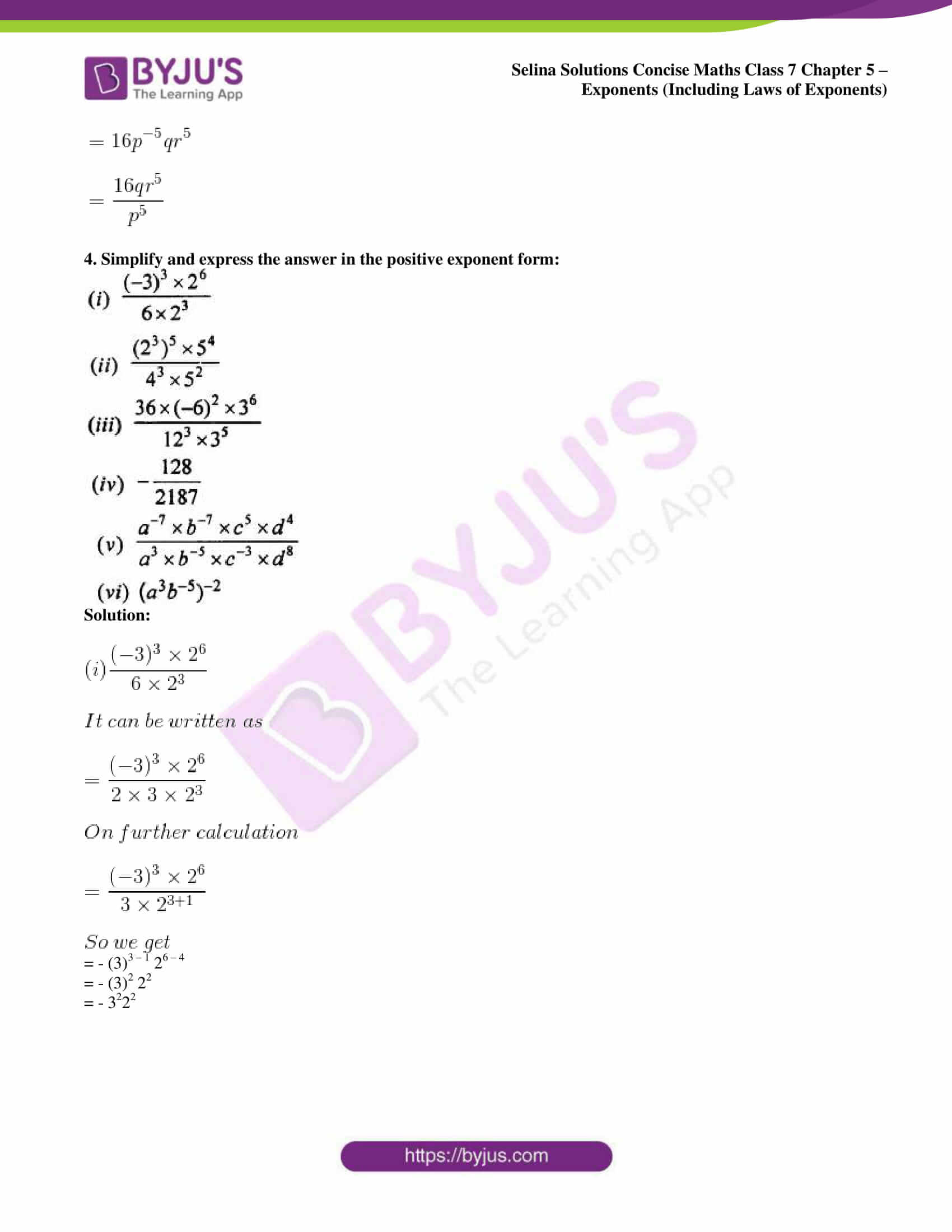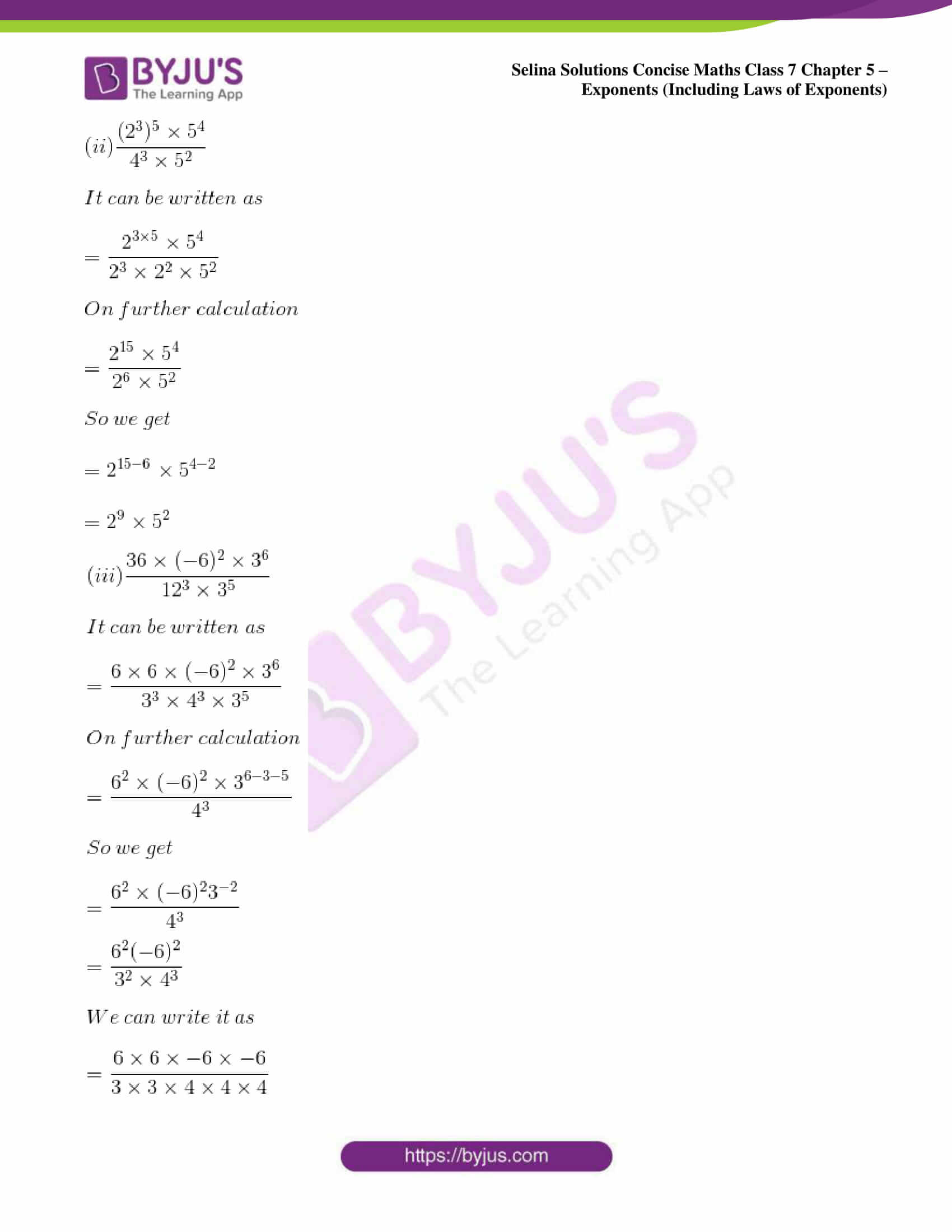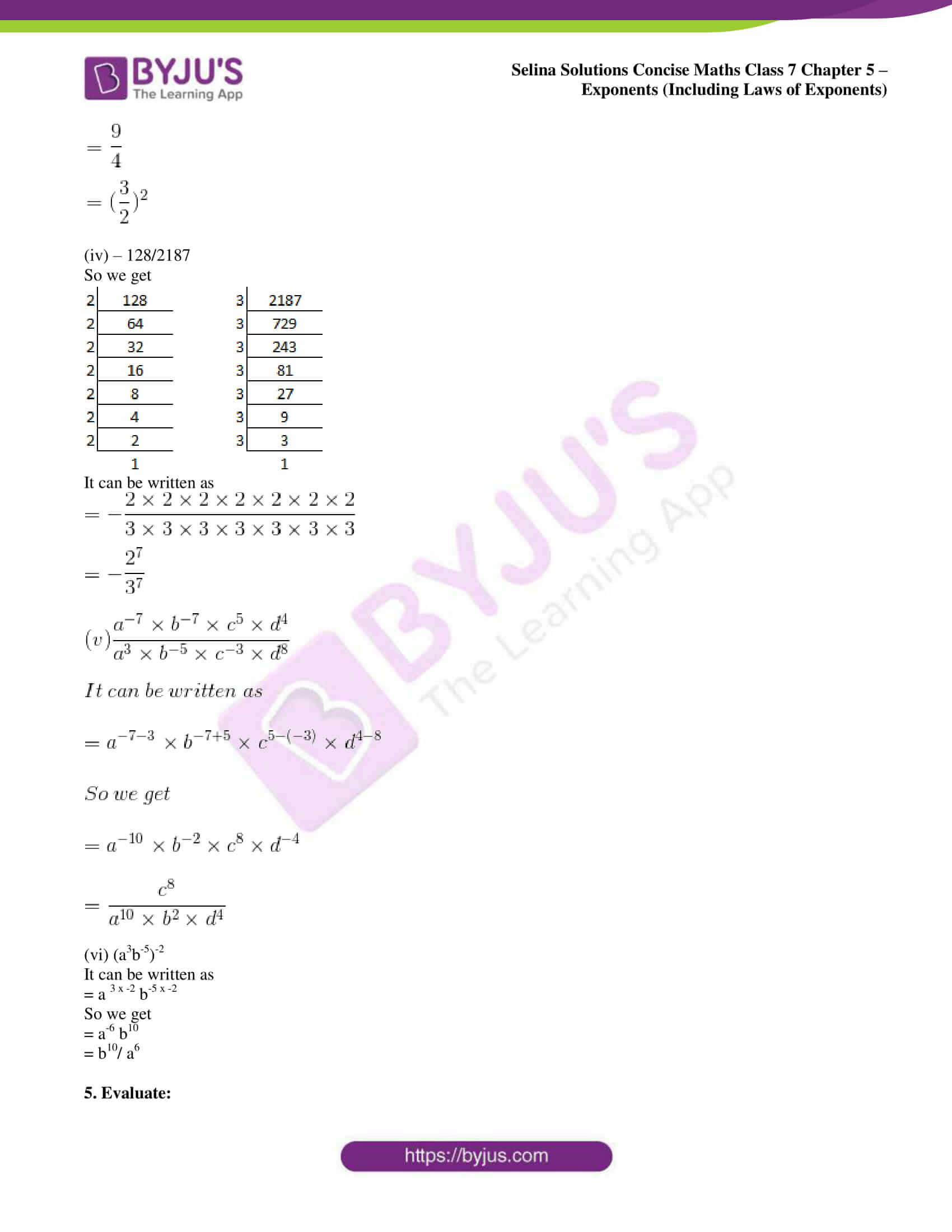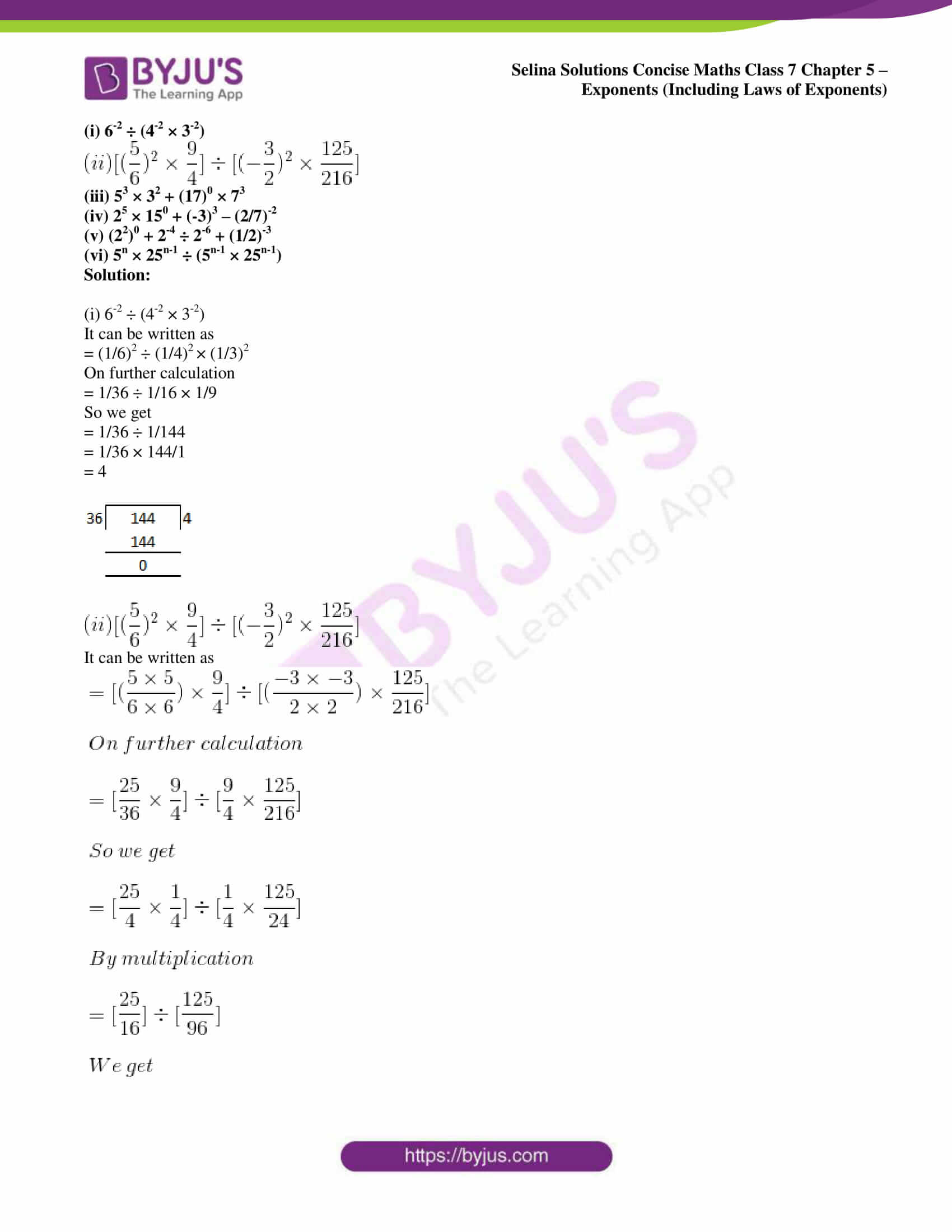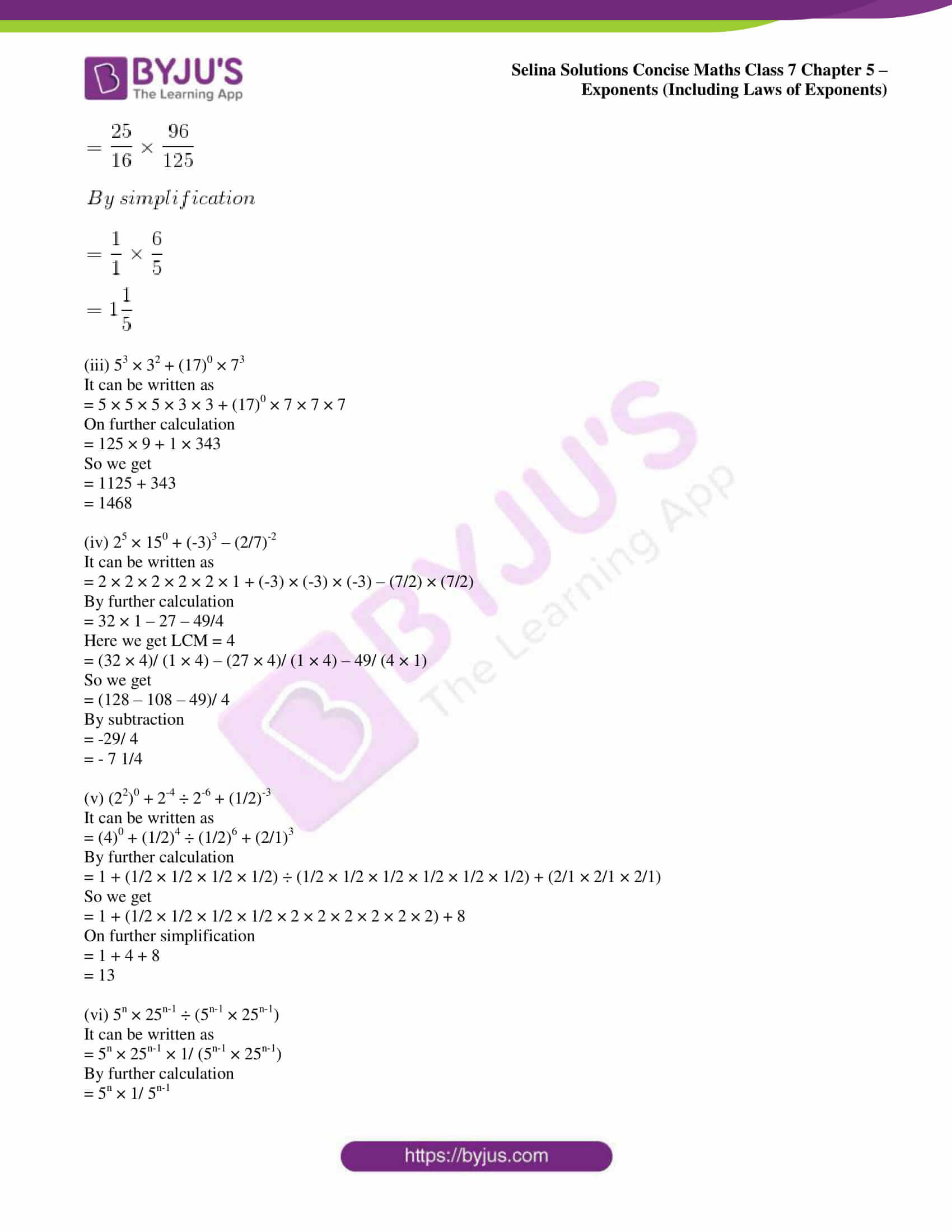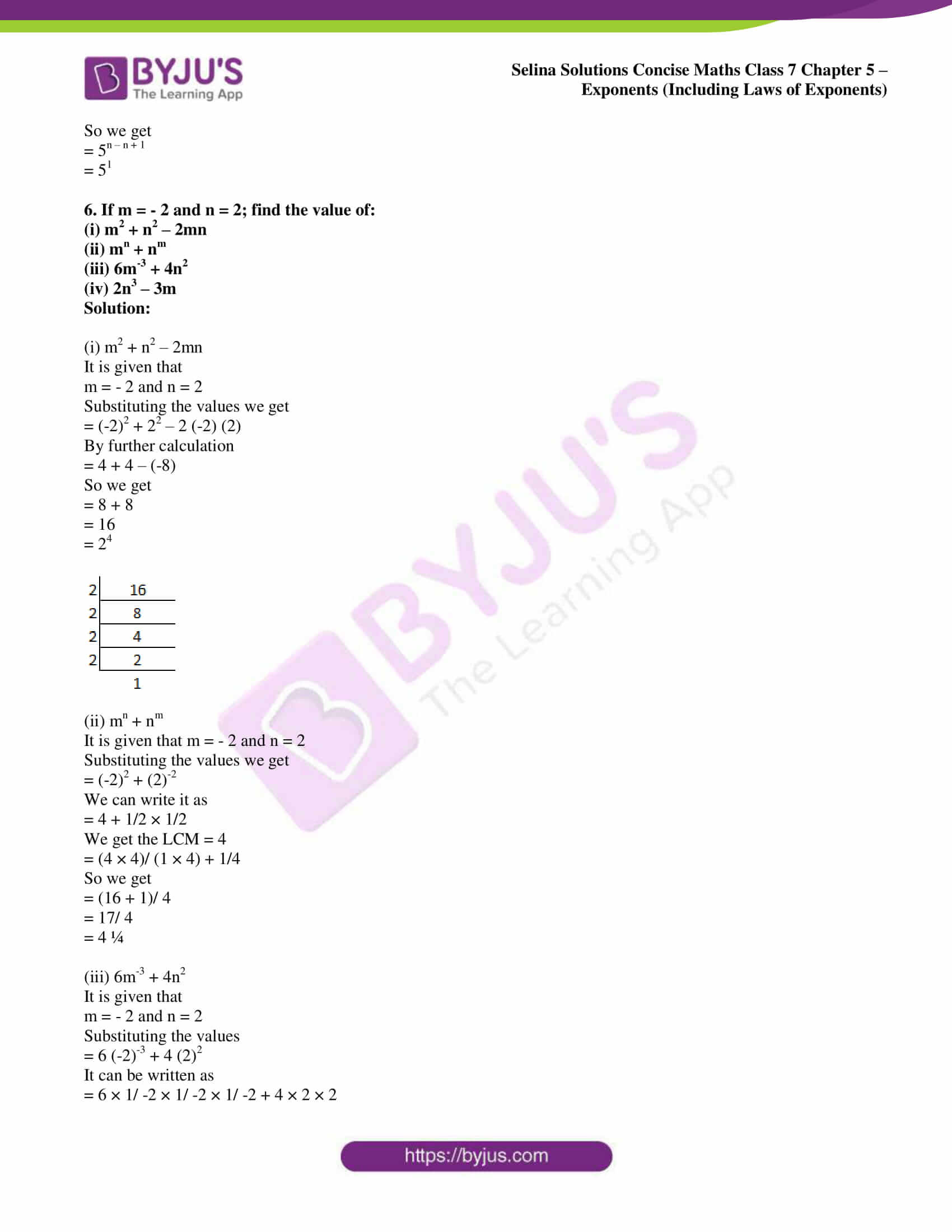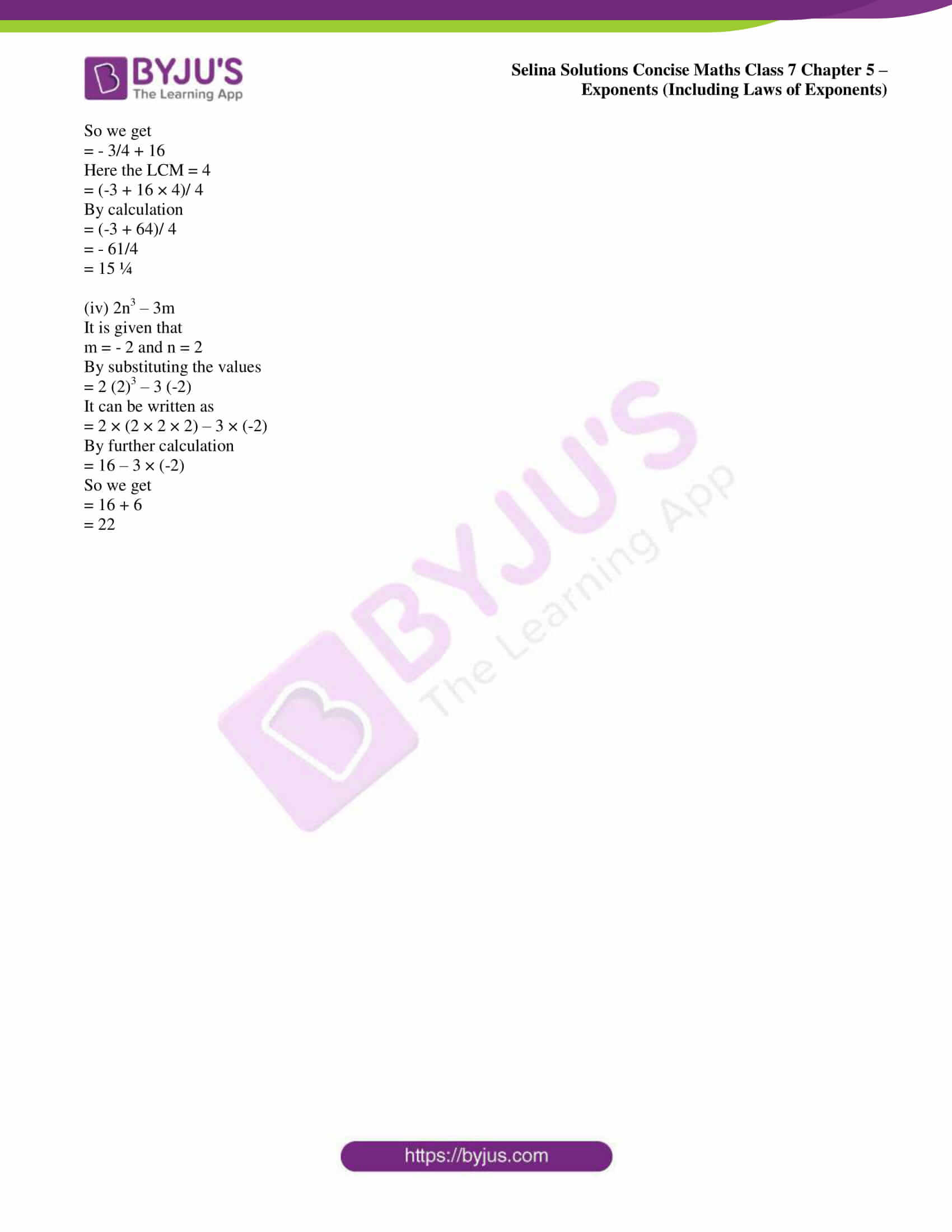## Access Selina Solutions Concise Maths Class 7 Chapter 5: Exponents (Including Laws of Exponents)

#### Exercise 5A page: 73

1. Find the value of:

(i) 62

(ii) 73

(iii) 44

(iv) 55

(v) 83

(vi) 75

Solution:

(i) 62

It can be written as

= 6 × 6

= 36

(ii) 73

It can be written as

= 7 × 7 × 7

= 343

(iii) 44

It can be written as

= 4 × 4 × 4 × 4

= 256

(iv) 55

It can be written as

= 5 × 5 × 5 × 5 × 5

= 3125

(v) 83

It can be written as

= 8 × 8 × 8

= 512

(vi) 75

It can be written as

= 7 × 7 × 7 × 7 × 7

= 16807

2. Evaluate:

(i) 23 × 42

(ii) 23 × 52

(iii) 33 × 52

(iv) 22 × 33

(v) 32 × 53

(vi) 53 × 24

(vii) 32 × 42

(viii) (4 × 3)3

(ix) (5 × 4)2

Solution:

(i) 23 × 42

It can be written as

= 2 × 2 × 2 × 4 × 4

On further calculation

= 8 × 16

= 128

(ii) 23 × 52

It can be written as

= 2 × 2 × 2 × 5 × 5

On further calculation

= 8 × 25

= 200

(iii) 33 × 52

It can be written as

= 3 × 3 × 3 × 5 × 5

On further calculation

= 27 × 25

= 675

(iv) 22 × 33

It can be written as

= 2 × 2 × 3 × 3 × 3

On further calculation

= 4 × 27

= 108

(v) 32 × 53

It can be written as

= 3 × 3 × 5 × 5 × 5

On further calculation

= 9 × 125

= 1125

(vi) 53 × 24

It can be written as

= 5 × 5 × 5 × 2 × 2 × 2 × 2

On further calculation

= 125 × 16

= 2000

(vii) 32 × 42

It can be written as

= 3 × 3 × 4 × 4

On further calculation

= 9 × 16

= 144

(viii) (4 × 3)3

It can be written as

= 4 × 4 × 4 × 3 × 3 × 3

On further calculation

= 64 × 27

= 1728

(ix) (5 × 4)2

It can be written as

= 5 × 5 × 4 × 4

On further calculation

= 25 × 16

= 400

3. Evaluate:

(i) (3/4)4

(ii) (-5/6)5

(iii) (-3/-5)3

Solution:

(i) (3/4)4

It can be written as

= (3/4) × (3/4) × (3/4) × (3/4)

On further calculation

= (3 × 3 × 3 × 3)/ (4 × 4 × 4 × 4)

= 81/256

(ii) (-5/6)5

It can be written as

= (-5/6) × (-5/6) × (-5/6) × (-5/6) × (-5/6)

On further calculation

= [(-5) × (-5) × (-5) × (-5) × (-5)]/ (6 × 6 × 6 × 6 × 6)

= -3125/776

(iii) (-3/-5)3

It can be written as

= (-3/-5) × (-3/-5) × (-3/-5)

On further calculation

= [(-3) × (-3) × (-3)]/ [(-5) × (-5) × (-5)]

= 27/125

4. Evaluate:

(i) (2/3)3 × (3/4)2

(ii) (-3/4)3 × (2/3)4

(iii) (3/5)2 × (-2/3)3

Solution:

(i) (2/3)3 × (3/4)2

It can be written as

= (2/3) × (2/3) × (2/3) × (3/4) × (3/4)

On further calculation

= 8/27 × 9/16

= 1/6

(ii) (-3/4)3 × (2/3)4

It can be written as

= (-3/4) × (-3/4) × (-3/4) × (2/3) × (2/3) × (2/3) × (2/3)

On further calculation

= -27/64 × 16/81

= -1/12

(iii) (3/5)2 × (-2/3)3

It can be written as

= (3/5) × (3/5) × (-2/3) × (-2/3) × (-2/3)

On further calculation

= 9/25 × (-8/27)

= -8/75

5. Which is greater:

(i) 23 or 32

(ii) 25 or 52

(iii) 43 or 34

(iv) 54 or 45

Solution:

(i) 23 or 32

It can be written as

23 = 2 × 2 × 2 = 8

32 = 3 × 3 = 9

Hence, 9 is greater than 8 i.e. 32 > 23.

(ii) 25 or 52

It can be written as

25 = 2 × 2 × 2 × 2 × 2 = 32

52 = 5 × 5 = 25

Hence, 32 is greater than 25 i.e. 25 > 52.

(iii) 43 or 34

It can be written as

43 = 4 × 4 × 4 = 64

34 = 3 × 3 × 3 × 3 = 81

Hence, 81 is greater than 64 i.e. 34 > 43.

(iv) 54 or 45

It can be written as

54 = 5 × 5 × 5 × 5 = 625

45 = 4 × 4 × 4 × 4 × 4 = 1024

Hence, 1024 is greater than 625 i.e. 45 > 54.

6. Express each of the following in exponential form:

(i) 512

(ii) 1250

(iii) 1458

(iv) 3600

(v) 1350

(vi) 1176

Solution:

(i) 512

It can be written as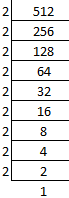So we get

512 = 2 × 2 × 2 × 2 × 2 × 2 × 2 × 2 × 2 = 29

(ii) 1250

It can be written as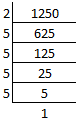So we get

1250 = 2 × 5 × 5 × 5 × 5 = 2 × 54

(iii) 1458

It can be written as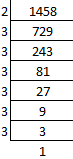So we get

1458 = 2 × 3 × 3 × 3 × 3 × 3 × 3 = 2 × 36

(iv) 3600

It can be written as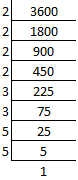So we get

3600 = 2 × 2 × 2 × 2 × 3 × 3 × 5 × 5 = 24 × 32 × 52

(v) 1350

It can be written as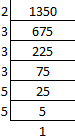So we get

1350 = 2 × 3 × 3 × 3 × 5 × 5 = 2 × 33 × 52

(vi) 1176

It can be written as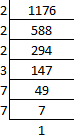So we get

1176 = 2 × 2 × 2 × 3 × 7 × 7 = 23 × 3 × 72

7. If a = 2 and b = 3, find the value of:

(i) (a + b)2

(ii) (b – a)3

(iii) (a × b)a

(iv) (a × b)b

Solution:

(i) (a + b)2

By substituting the values of a and b

= (2 + 3)2

On further calculation

= 52

= 5 × 5

= 25

(ii) (b – a)3

By substituting the values of a and b

= (3 – 2)3

On further calculation

= 13

= 1 × 1 × 1

= 1

(iii) (a × b)a

By substituting the values of a and b

= (2 × 3)2

On further calculation

= 62

= 6 × 6

= 36

(iv) (a × b)b

By substituting the values of a and b

= (2 × 3)3

On further calculation

= 63

= 6 × 6 × 6

= 216

8. Express:

(i) 1024 as a power of 2.

(ii) 343 as a power of 7.

(iii) 729 as a power of 3.

Solution:

(i) 1024 as a power of 2.

It can be written as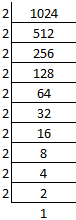So we get

1024 = 2 × 2 × 2 × 2 × 2 × 2 × 2 × 2 × 2 × 2 = 210

(ii) 343 as a power of 7.

It can be written as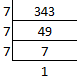So we get

343 = 7 × 7 × 7 = 73

(iii) 729 as a power of 3.

It can be written as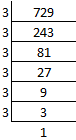So we get

729 = 3 × 3 × 3 × 3 × 3 × 3 = 36

9. If 27 × 32 = 3x × 2y; find the values of x and y.

Solution:

It is given that

27 × 32 = 3x × 2y

So we get

27 = 3x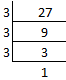Here

27 = 3 × 3 × 3 = 33 = 3x

We get

x = 3x

Similarly

32 = 2y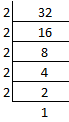Here

32 = 2 × 2 × 2 × 2 × 2 = 25 = 2y

We get

y = 5

10. If 64 × 625 = 2a × 5b; find: (i) the values of a and b. (ii) 2b × 5a.

Solution:

(i) the values of a and b

It is given that

64 × 625 = 2a × 5b

We know that

64 = 2a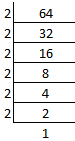We can write it as

64 = 2 × 2 × 2 × 2 × 2 × 2

So we get

64 = 26

a = 6

Similarly

625 = 5b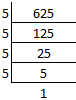We can write it as

625 = 5 × 5 × 5 × 5

So we get

625 = 54

b = 4

(ii) 2b × 5a

Substituting the values of a and b

= 24 × 56

It can be written as

= 2 × 2 × 2 × 2 × 5 × 5 × 5 × 5 × 5 × 5

So we get

= 16 × 15625

= 250000

#### Exercise 5B page: 75

1. Fill in the blanks:

(i) In 52 = 25, base = ………. and index = …………

(ii) If index = 3x and base = 2y, the number = ………

Solution:

(i) In 52 = 25, base = 5 and index = 2.

(ii) If index = 3x and base = 2y, the number = 2y3x.

2. Evaluate:

(i) 28 ÷ 23
(ii) 2 28
(iii) (26)0
(iv) (3o)6
(v) 83 x 8-5 x 84
(vi) 5x 53 ÷ 55
(vii) 54 ÷ 53 x 55
(viii) 44 ÷ 43 x 40
(ix) (35 x 47 x 58)0

Solution:

(i) 28 ÷ 23

It can be written as

= 28/ 23

On further calculation

= 2 8-3

= 25

(ii) 2 28

It can be written as

= 23/ 28

On further calculation

= 2 3-8

So we get

= 2 -5

= 1/25

(iii) (26)0

It can be written as

= 2 6 × 0

On further calculation

= 20

So we get

= 1

(iv) (3o) 6

It can be written as

= 3 0 × 6

On further calculation

= 30

So we get

= 1

(v) 83 x 8-5 x 84

It can be written as

= 8 3 + 4 – 5

On further calculation

= 8 7 – 5

So we get

= 82

(vi) 5x 53 ÷ 55

It can be written as

= (5x 53)/ 55

On further calculation

= 5 4 + 3 – 5

So we get

= 5 7 – 5

= 52

(vii) 54 ÷ 53 x 55

It can be written as

= 54/ 53 x 55

On further calculation

= 5 4 – 3 + 5

So we get

= 56

(viii) 44 ÷ 43 x 40

It can be written as

= 44/ (43 x 40)

On further calculation

= 44/ (43 x 1)

So we get

= 44/43

= 44-3

= 41

= 4

(ix) (35 x 47 x 58)0

It can be written as

= 35 × 0 × 47 × 0 × 58 × 0

On further calculation

= 30 × 40 × 50

So we get

= 1 × 1 × 1

= 1

3. Simplify, giving answers with positive index:

(i) 2b6. b3. 5b4

(ii) x2y3. 6x5y. 9x3y4

(iii) (-a)5 (a2)

(iv) (-y)2 (-y)3

(v) (-3)2 (3)3

(vi) (-4x) (-5x2)

(vii) (5a2b) (2ab2) (a3b)

(viii) x2a + 7. x2a – 8

(ix) 3y. 32. 3-4

(x) 24a. 23a. 2-a

(xi) 4x2y2 ÷ 9x3y3

(xii) (102)3 (x8)12

(xiii) (a10)10 (16)10

(xiv) (n2)2 (-n2)3

(xv) – (3ab)2 (-5a2bc4)2

(xvi) (-2)2 × (0)3 × (3)3

(xvii) (2a3)4 (4a2)2

(xviii) (4x2y3)3 ÷ (3x2y3)3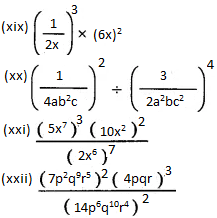Solution:

(i) 2b6. b3. 5b4

It can be written as

= 2 × 5 × b6 + 3 + 4

On further calculation

= 10 b13

(ii) x2y3. 6x5y. 9x3y4

It can be written as

= 6 × 9 × x2 + 5 + 3 × y3 + 1 + 4

On further calculation

= 54 x10 y8

(iii) (-a)5 (a2)

It can be written as

= (-1 × a)5 × a2

On further calculation

= (-1)5 × a5 + 2

So we get

= – 1 × a7

= – a7

(iv) (-y)2 (-y)3

It can be written as

= (-1 × y)2. (-1 × y)3

On further calculation

= (-1)2. y2. (-1)3 × y3

So we get

= 12 + 3. y2 + 3

= 15 y5

= y5

(v) (-3)2 (3)3

It can be written as

= (-1 × 3)2. (3)3

On further calculation

= (-1)2 × 32. 33

So we get

= (-1)2. 32 + 3

= 1. 35

= 35

(vi) (-4x) (-5x2)

It can be written as

= (-1 × 4 × x). (-1 × 5 × x2)1

On further calculation

= (- 1 × 4 × x). (-1 × 5 × x2)

So we get

= – 1 × – 1 × 4 × 5 × x1 + 2

Here

= – 11 + 1. 41. 51 x3

= 20 x3

(vii) (5a2b) (2ab2) (a3b)

It can be written as

= 5 × 2 × a2 + 1 + 3 × b1 + 2 + 1

On further calculation

= 10 a6b4

(viii) x2a + 7. x2a – 8

It can be written as

= x2a + 7 + 2a – 8

On further calculation

= x4a – 1

(ix) 3y. 32. 3-4

It can be written as

= 3y. 32/34

On further calculation

= 3y. (3 × 3)/ (3 × 3 × 3 × 3)

So we get

= 3y × 1/32

= 3y – 2

(x) 24a. 23a. 2-a

It can be written as

= 2 4a + 3a – a

On further calculation

= 2 7a – a

So we get

= 26a

(xi) 4x2y2 ÷ 9x3y3

It can be written as

= 4x2y2/ 9x3y3

On further calculation

= 4x2 – 3 y2 – 3/ 9

So we get

= 4x-1y-1/ 9

= 4/9xy

(xii) (102)3 (x8)12

It can be written as

= 102 × 3. x8 × 12

On further calculation

= 106 x96

(xiii) (a10)10 (16)10

It can be written as

= a10 × 10. 16 × 10

On further calculation

= a100. 160

So we get

= a100

(xiv) (n2)2 (-n2)3

It can be written as

= n2 × 2. (-n)2 × 3

On further calculation

= n4. (-n)6

So we get

= – n4 – 16 n6

= – n4 + 6

= – n 10

(xv) – (3ab)2 (-5a2bc4)2

It can be written as

= – (32a2b2) × (-1)2 × 52a2 × 2b2c4 × 2

On further calculation

= – (32a2b2) (52a4b2c8)

So we get

= – 32. 52. a2 + 4 b2 + 2 c8

= – 225a6b4c8

(xvi) (-2)2 × (0)3 × (3)3

It can be written as

= 4 × 0 × 27

On further calculation

= 0

(xvii) (2a3)4 (4a2)2

It can be written as

= (2a3)4 (22a2)2

On further calculation

= 24 a3 × 4. 22 × 2. a2 × 2

So we get

= 24 a12. 24 a4

Here

= 24 + 4. a12 + 4

= 28 a16

We get

= 2 × 2 × 2 × 2 × 2 × 2 × 2 × 2 × a16

= 256 a16

(xviii) (4x2y3)3 ÷ (3x2y3)3

It can be written as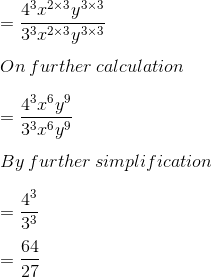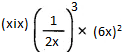It can be written as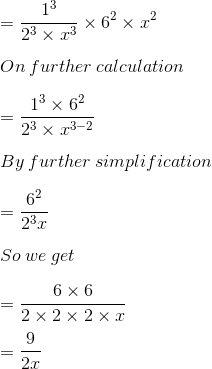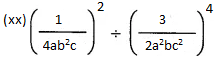It can be written as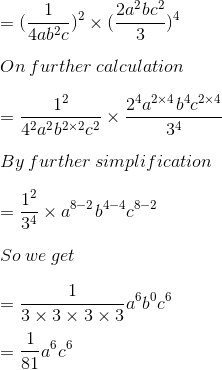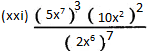It can be written as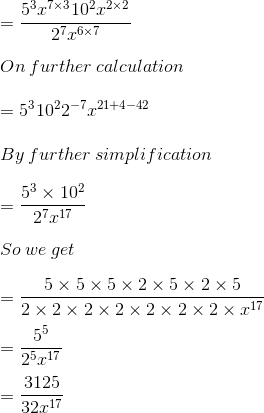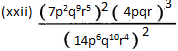It can be written as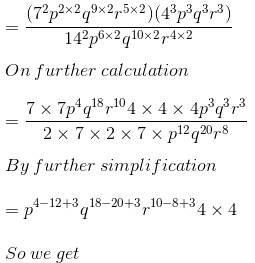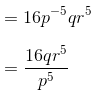4. Simplify and express the answer in the positive exponent form: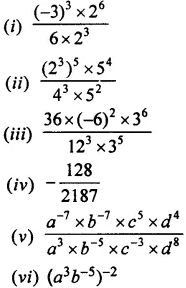Solution: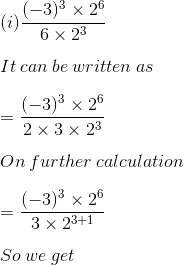= – (3)3 – 1 26 – 4

= – (3)2 22

= – 3222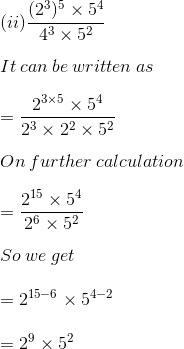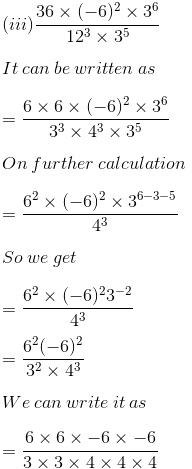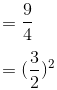(iv) – 128/2187

So we get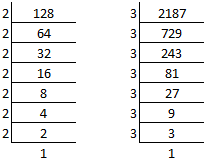It can be written as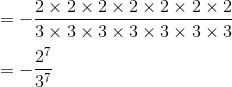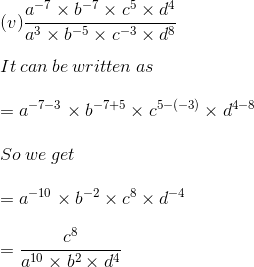(vi) (a3b-5)-2

It can be written as

= a 3 x -2 b-5 x -2

So we get

= a-6 b10

= b10/ a6

5. Evaluate:

(i) 6-2 ÷ (4-2 × 3-2)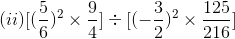(iii) 53 × 32 + (17)0 × 73

(iv) 25 × 150 + (-3)3 – (2/7)-2

(v) (22)0 + 2-4 ÷ 2-6 + (1/2)-3

(vi) 5n × 25n-1 ÷ (5n-1 × 25n-1)

Solution:

(i) 6-2 ÷ (4-2 × 3-2)

It can be written as

= (1/6)2 ÷ (1/4)2 × (1/3)2

On further calculation

= 1/36 ÷ 1/16 × 1/9

So we get

= 1/36 ÷ 1/144

= 1/36 × 144/1

= 4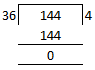It can be written as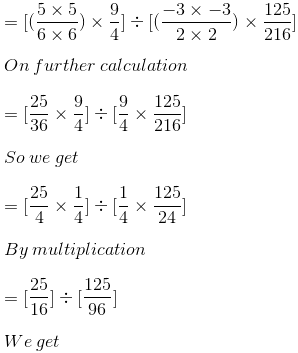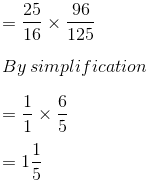(iii) 53 × 32 + (17)0 × 73

It can be written as

= 5 × 5 × 5 × 3 × 3 + (17)0 × 7 × 7 × 7

On further calculation

= 125 × 9 + 1 × 343

So we get

= 1125 + 343

= 1468

(iv) 25 × 150 + (-3)3 – (2/7)-2

It can be written as

= 2 × 2 × 2 × 2 × 2 × 1 + (-3) × (-3) × (-3) – (7/2) × (7/2)

By further calculation

= 32 × 1 – 27 – 49/4

Here we get LCM = 4

= (32 × 4)/ (1 × 4) – (27 × 4)/ (1 × 4) – 49/ (4 × 1)

So we get

= (128 – 108 – 49)/ 4

By subtraction

= -29/ 4

= – 7 1/4

(v) (22)0 + 2-4 ÷ 2-6 + (1/2)-3

It can be written as

= (4)0 + (1/2)4 ÷ (1/2)6 + (2/1)3

By further calculation

= 1 + (1/2 × 1/2 × 1/2 × 1/2) ÷ (1/2 × 1/2 × 1/2 × 1/2 × 1/2 × 1/2) + (2/1 × 2/1 × 2/1)

So we get

= 1 + (1/2 × 1/2 × 1/2 × 1/2 × 2 × 2 × 2 × 2 × 2 × 2) + 8

On further simplification

= 1 + 4 + 8

= 13

(vi) 5n × 25n-1 ÷ (5n-1 × 25n-1)

It can be written as

= 5n × 25n-1 × 1/ (5n-1 × 25n-1)

By further calculation

= 5n × 1/ 5n-1

So we get

= 5n – n + 1

= 51

6. If m = – 2 and n = 2; find the value of:

(i) m2 + n2 – 2mn

(ii) mn + nm

(iii) 6m-3 + 4n2

(iv) 2n3 – 3m

Solution:

(i) m2 + n2 – 2mn

It is given that

m = – 2 and n = 2

Substituting the values we get

= (-2)2 + 22 – 2 (-2) (2)

By further calculation

= 4 + 4 – (-8)

So we get

= 8 + 8

= 16

= 24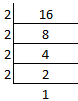(ii) mn + nm

It is given that m = – 2 and n = 2

Substituting the values we get

= (-2)2 + (2)-2

We can write it as

= 4 + 1/2 × 1/2

We get the LCM = 4

= (4 × 4)/ (1 × 4) + 1/4

So we get

= (16 + 1)/ 4

= 17/ 4

= 4 ¼

(iii) 6m-3 + 4n2

It is given that

m = – 2 and n = 2

Substituting the values

= 6 (-2)-3 + 4 (2)2

It can be written as

= 6 × 1/ -2 × 1/ -2 × 1/ -2 + 4 × 2 × 2

So we get

= – 3/4 + 16

Here the LCM = 4

= (-3 + 16 × 4)/ 4

By calculation

= (-3 + 64)/ 4

= – 61/4

= 15 ¼

(iv) 2n3 – 3m

It is given that

m = – 2 and n = 2

By substituting the values

= 2 (2)3 – 3 (-2)

It can be written as

= 2 × (2 × 2 × 2) – 3 × (-2)

By further calculation

= 16 – 3 × (-2)

So we get

= 16 + 6

= 22